# Selina Solutions Concise Mathematics Class 6 Chapter 19: Fundamental Operations

Selina Solutions Concise Mathematics Class 6 Chapter 19 Fundamental Operations has accurate answers, designed by subject matter experts at BYJU’S, in accordance with the grasping abilities of students. The solutions play a key role in improving students’ problem solving abilities in a short duration. Those who aim to achieve high marks in the annual examination are suggested to solve the textbook questions using these solutions. In order to obtain more conceptual knowledge, students can download Selina Solutions Concise Mathematics Class 6 Chapter 19 Fundamental Operations PDF, from the below mentioned links.

Chapter 19 provides in-depth knowledge relying on Fundamental Operations such as addition, subtraction, multiplication and division. Students who practice these solutions on a regular basis, learn multiple ways of solving complex problems effortlessly.

## Selina Solutions Concise Mathematics Class 6 Chapter 19: Fundamental Operations Download PDF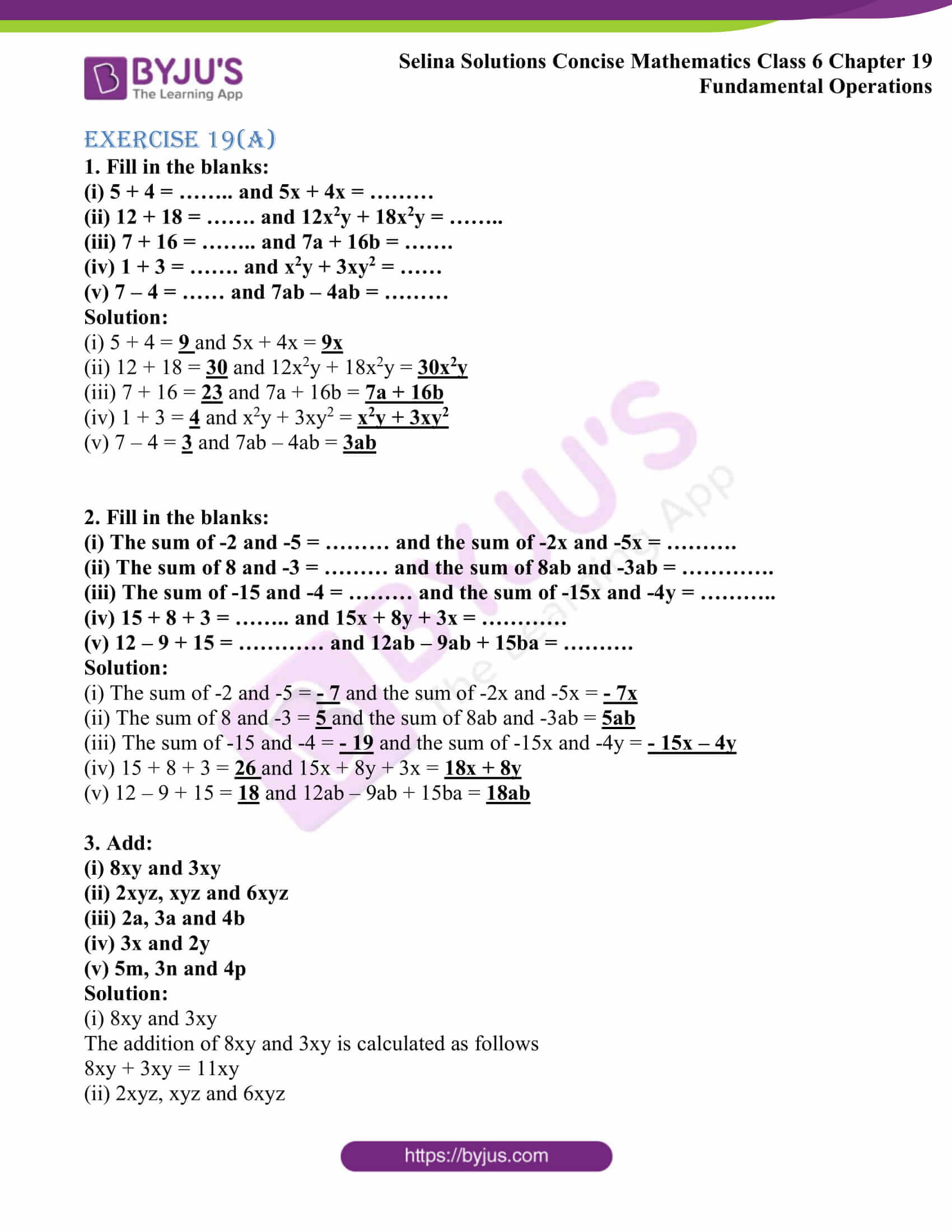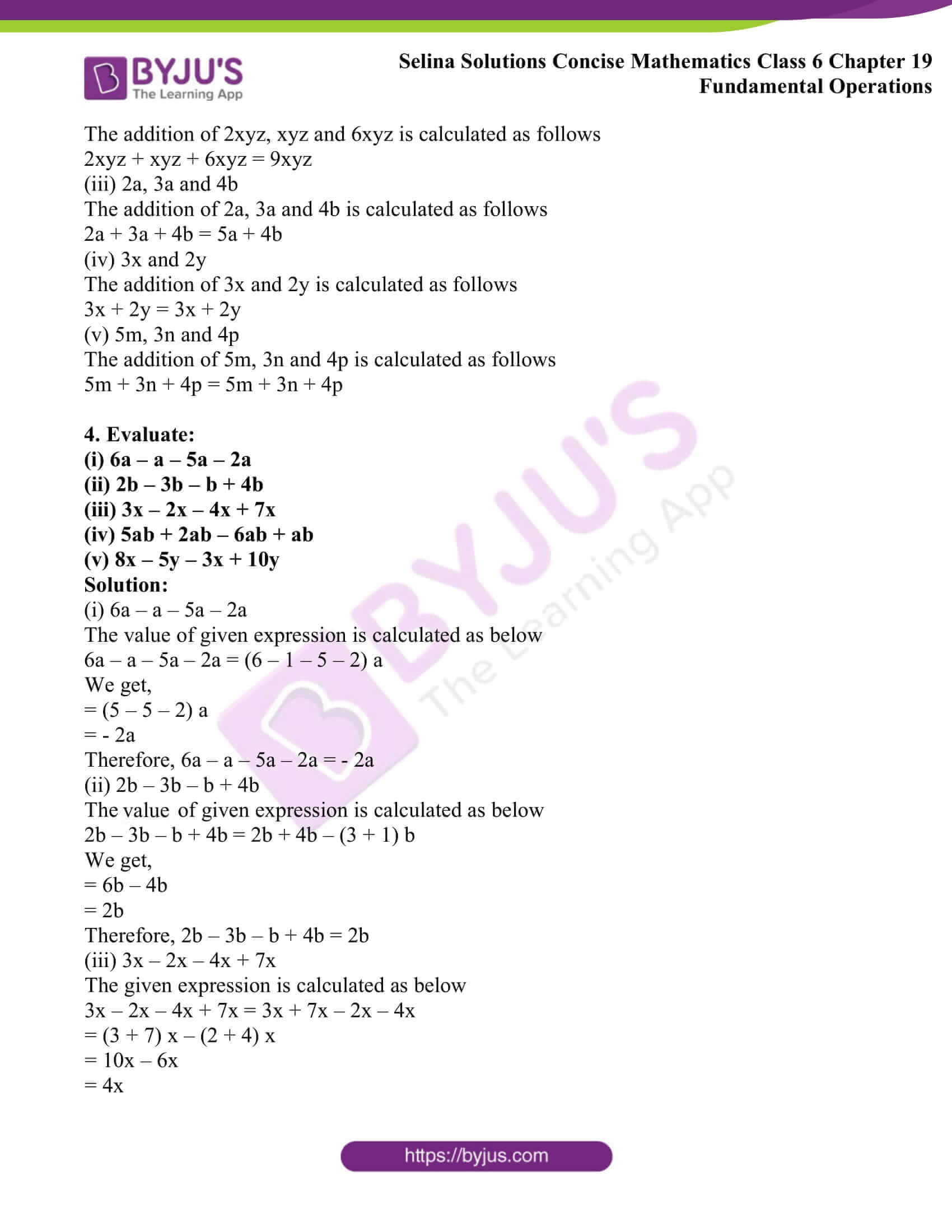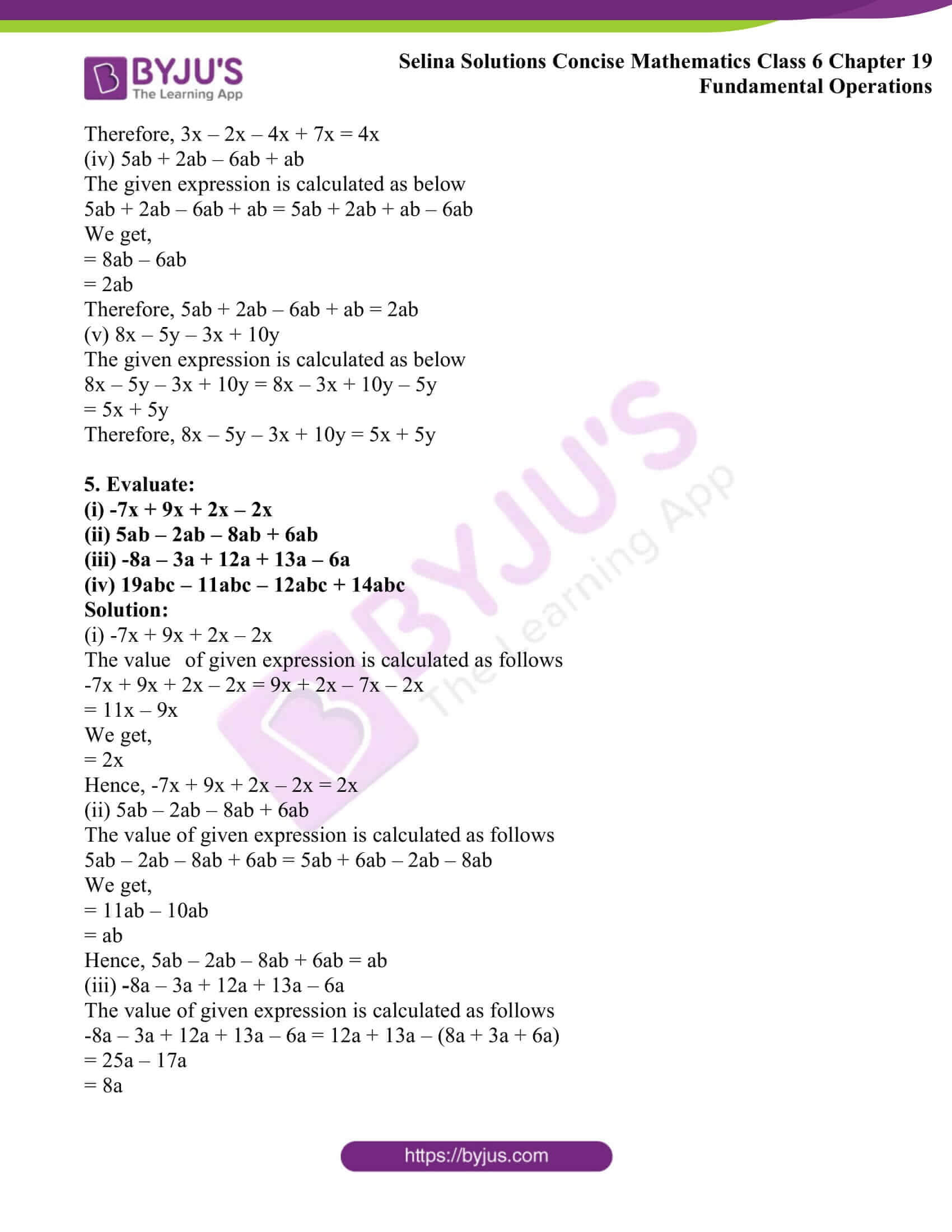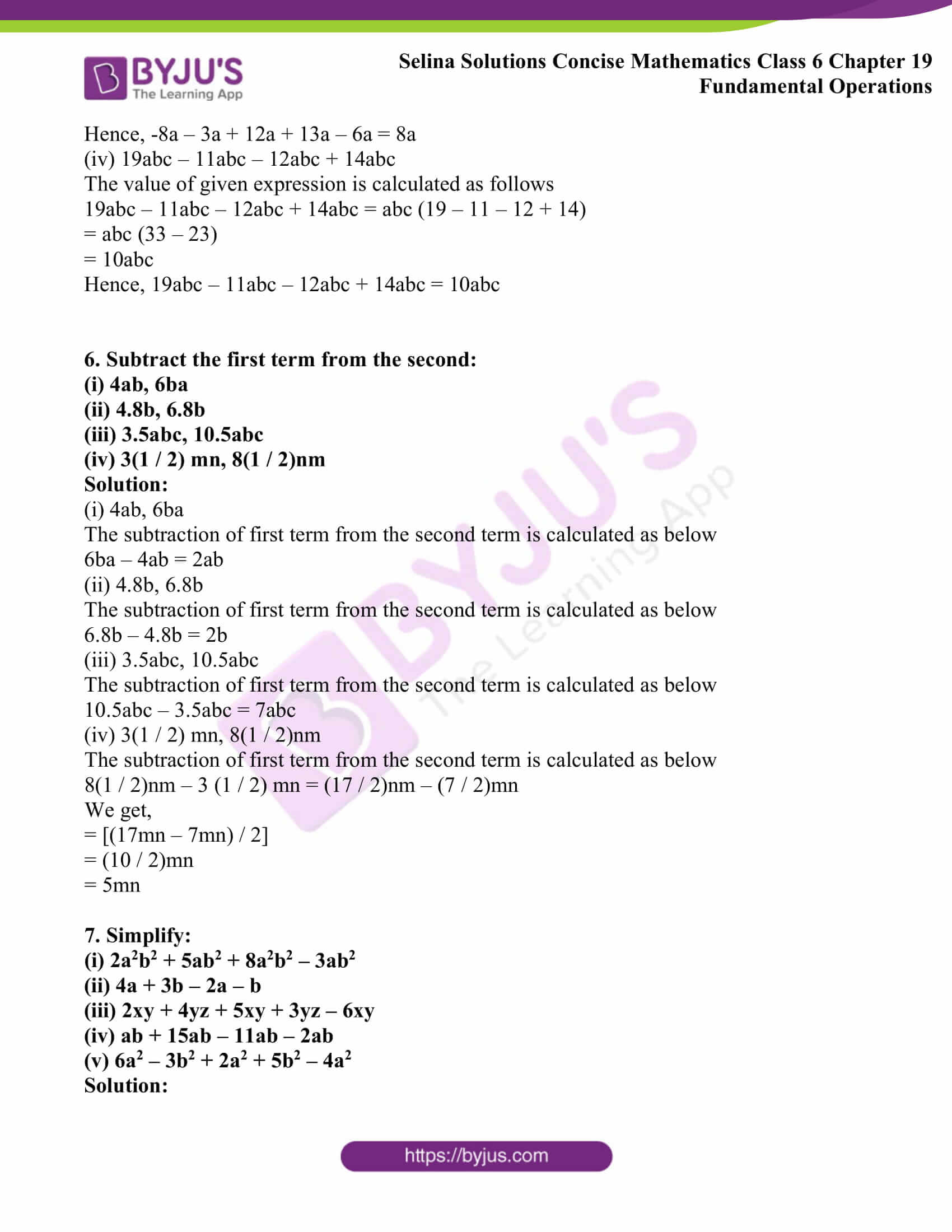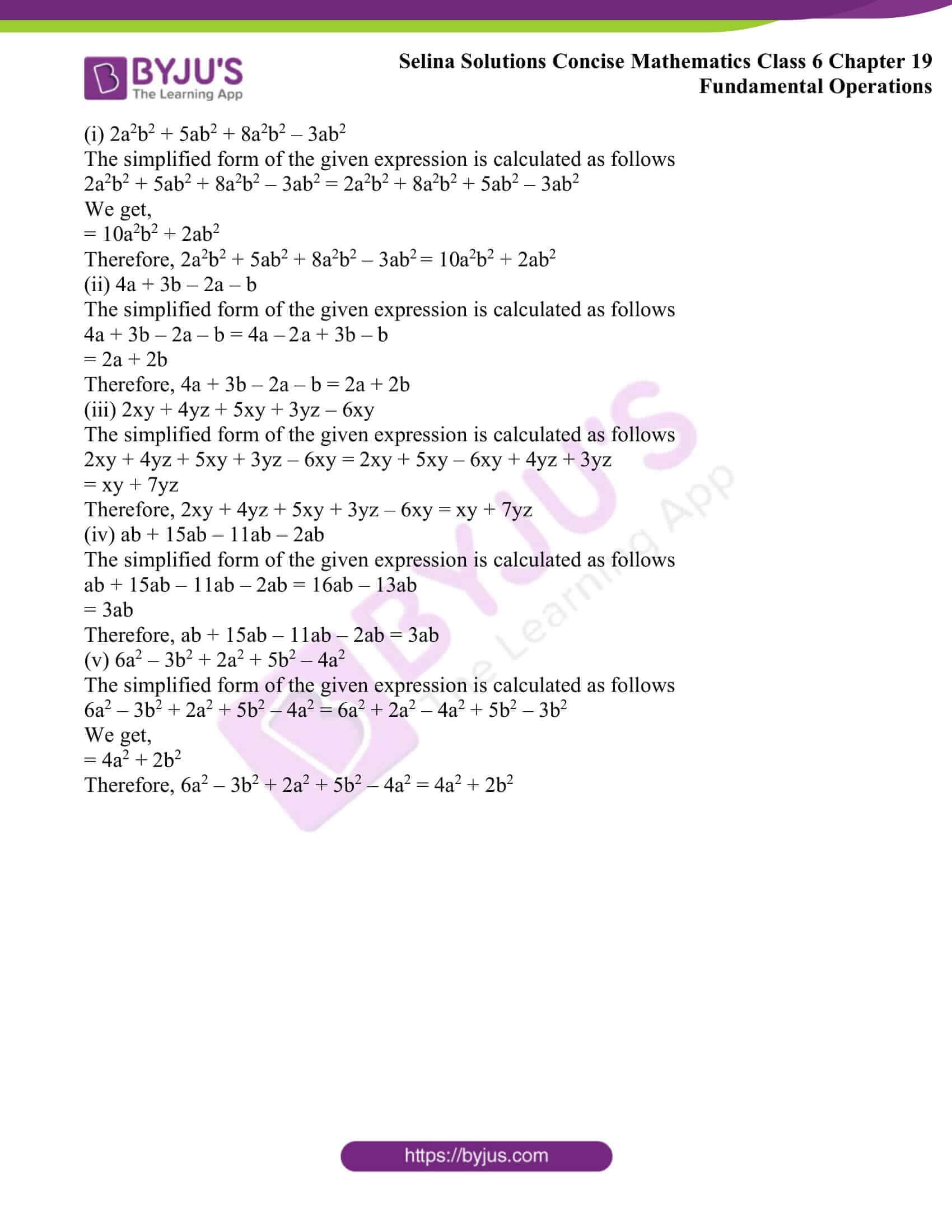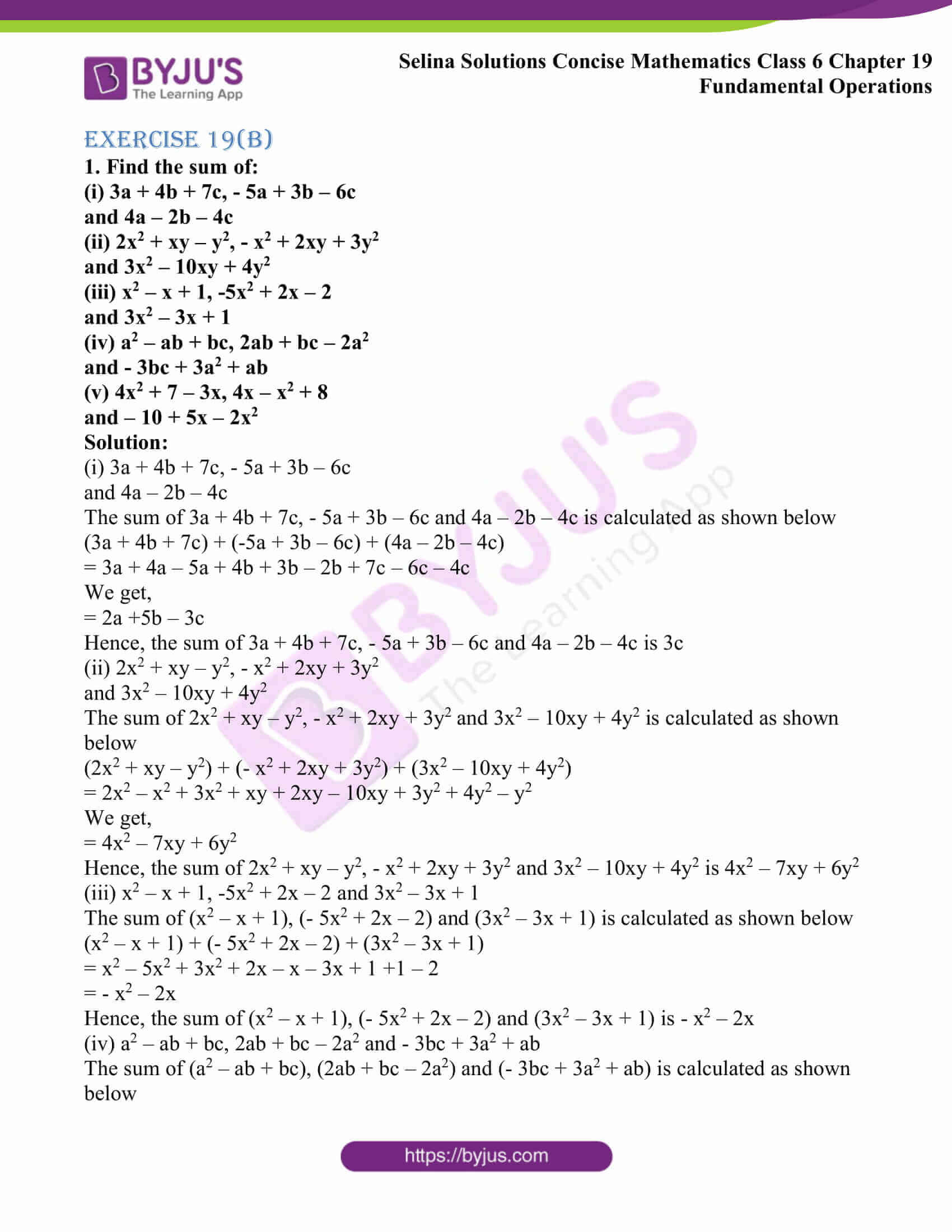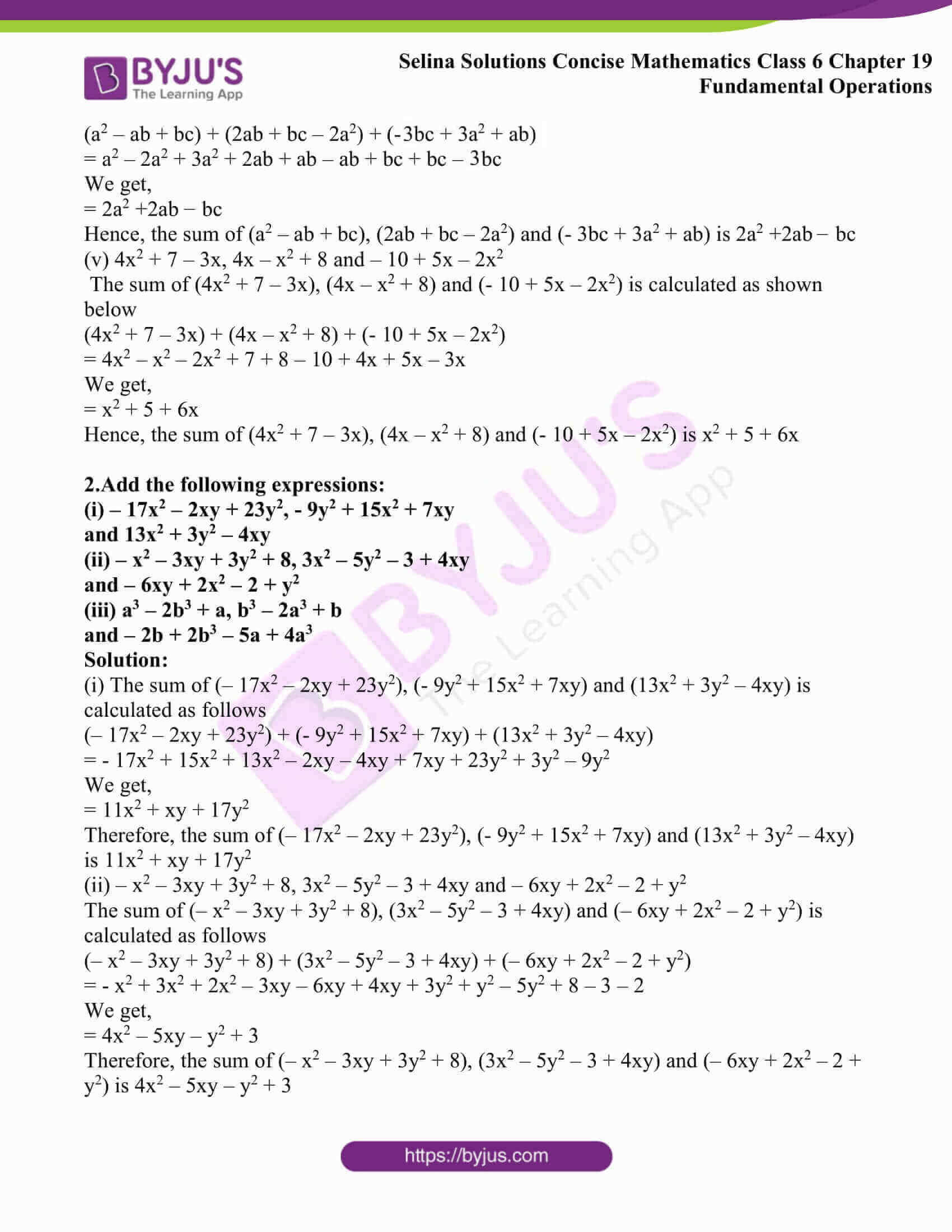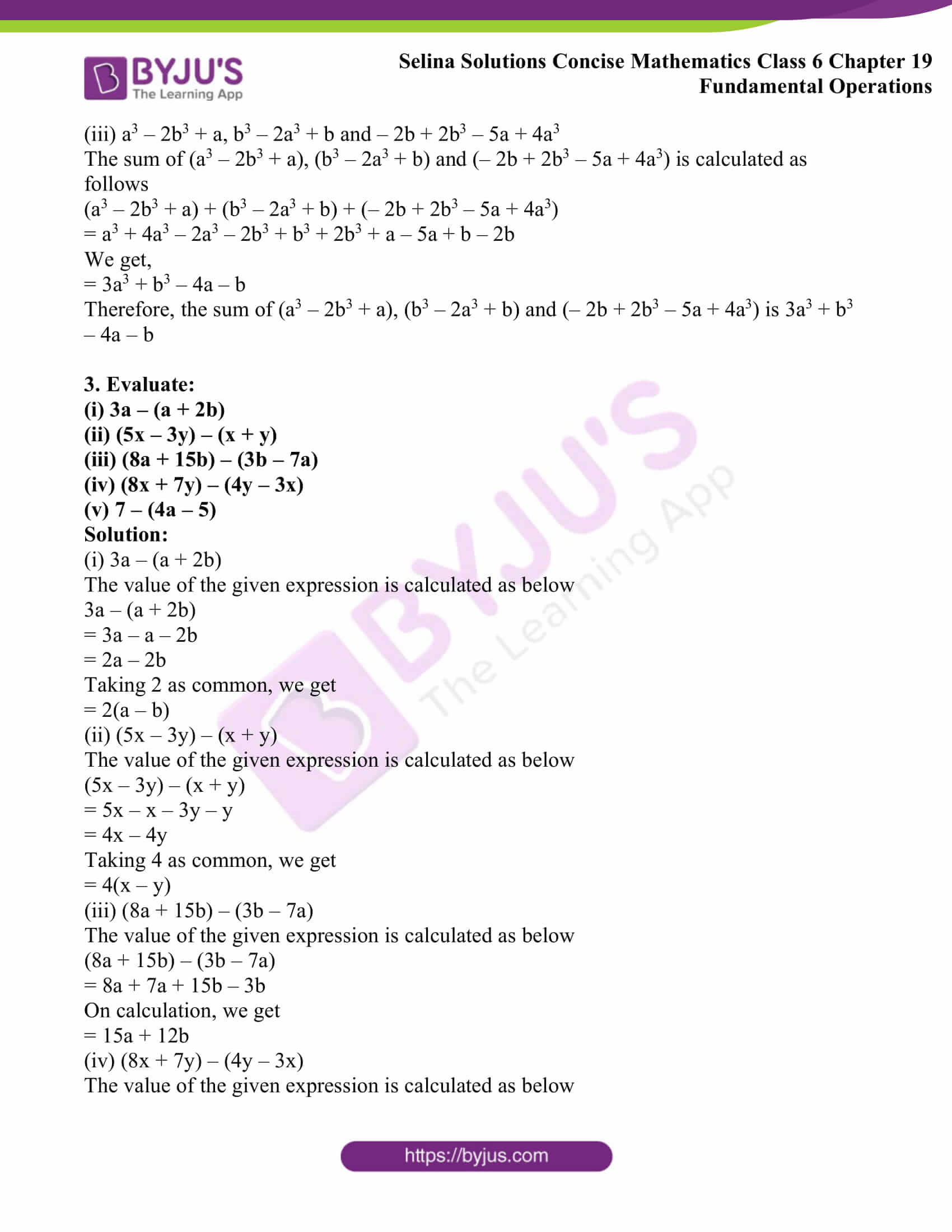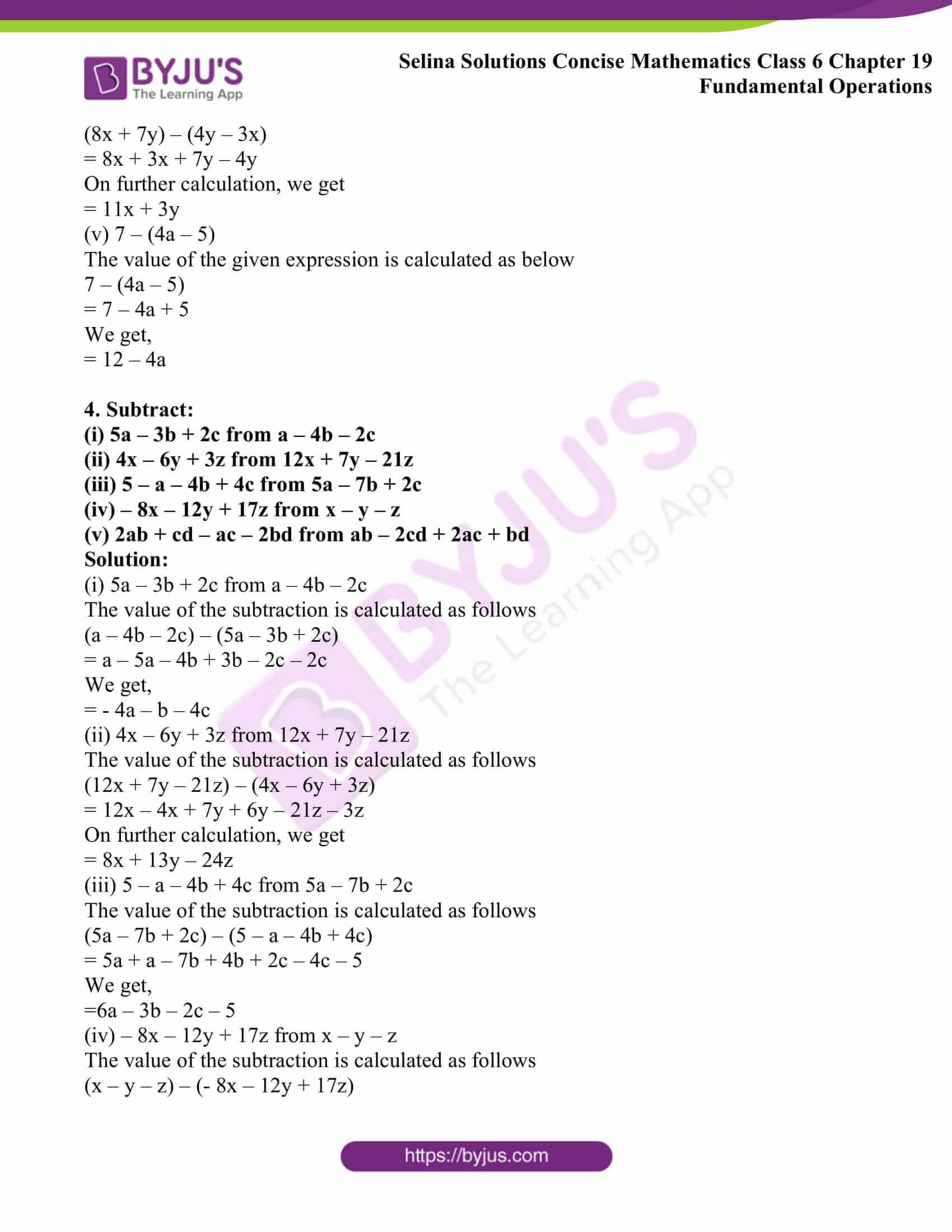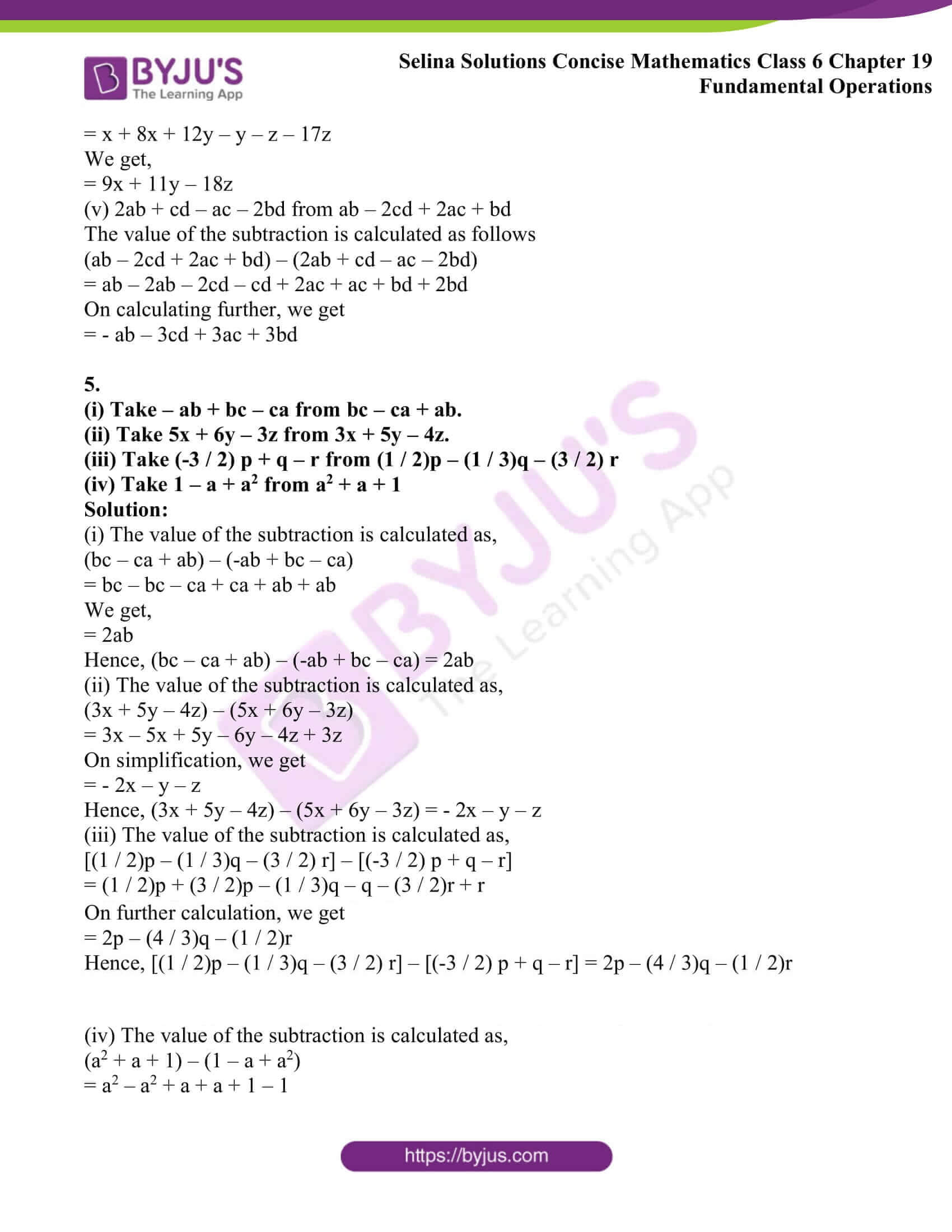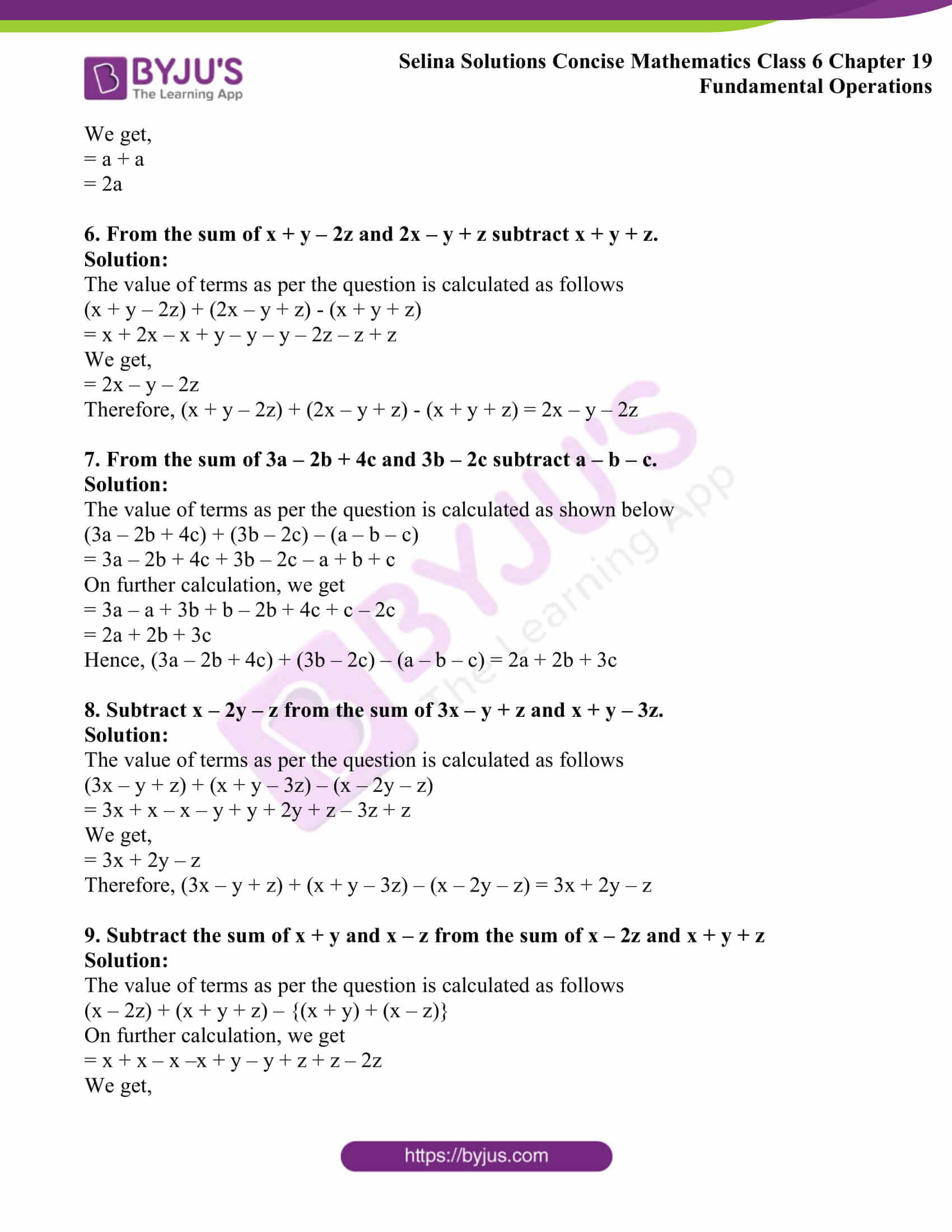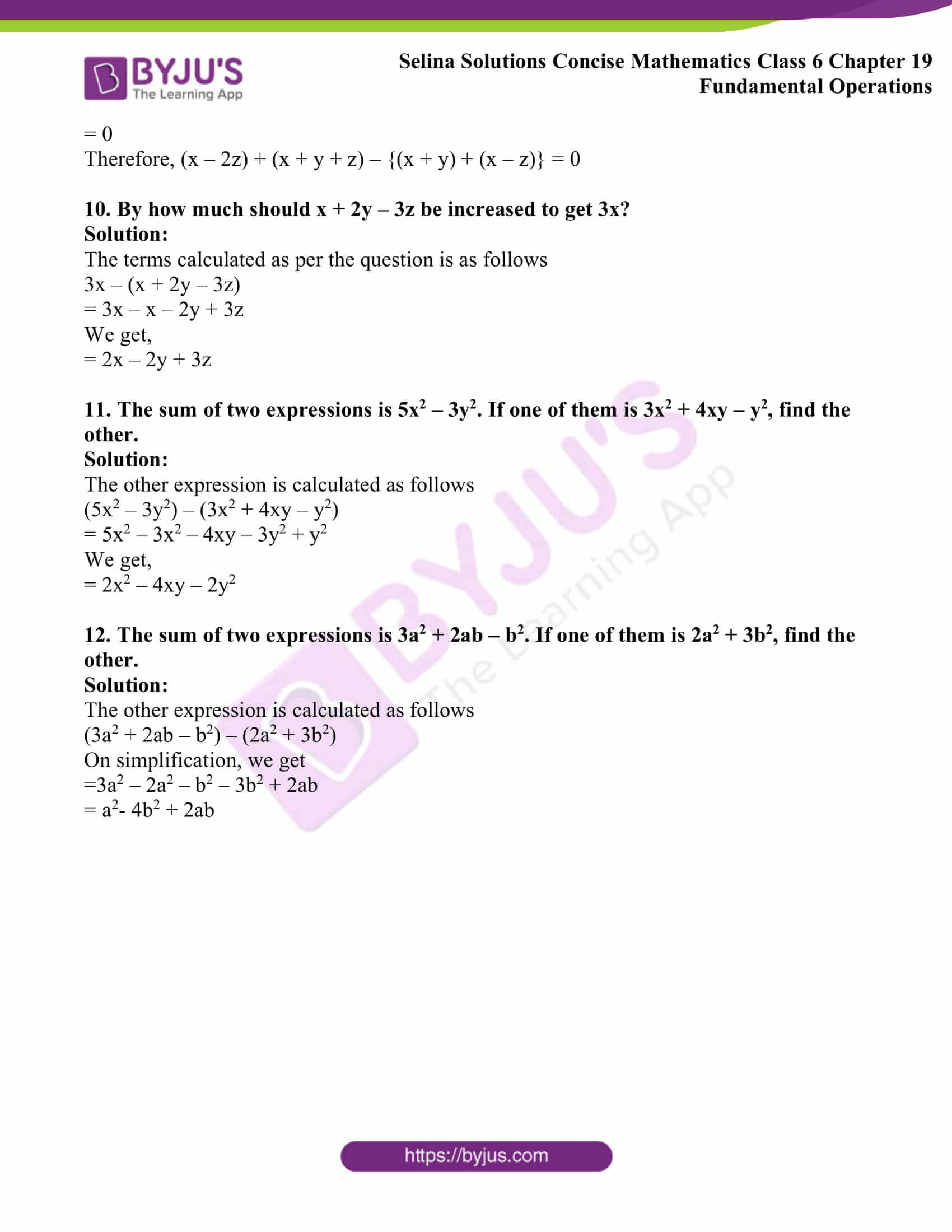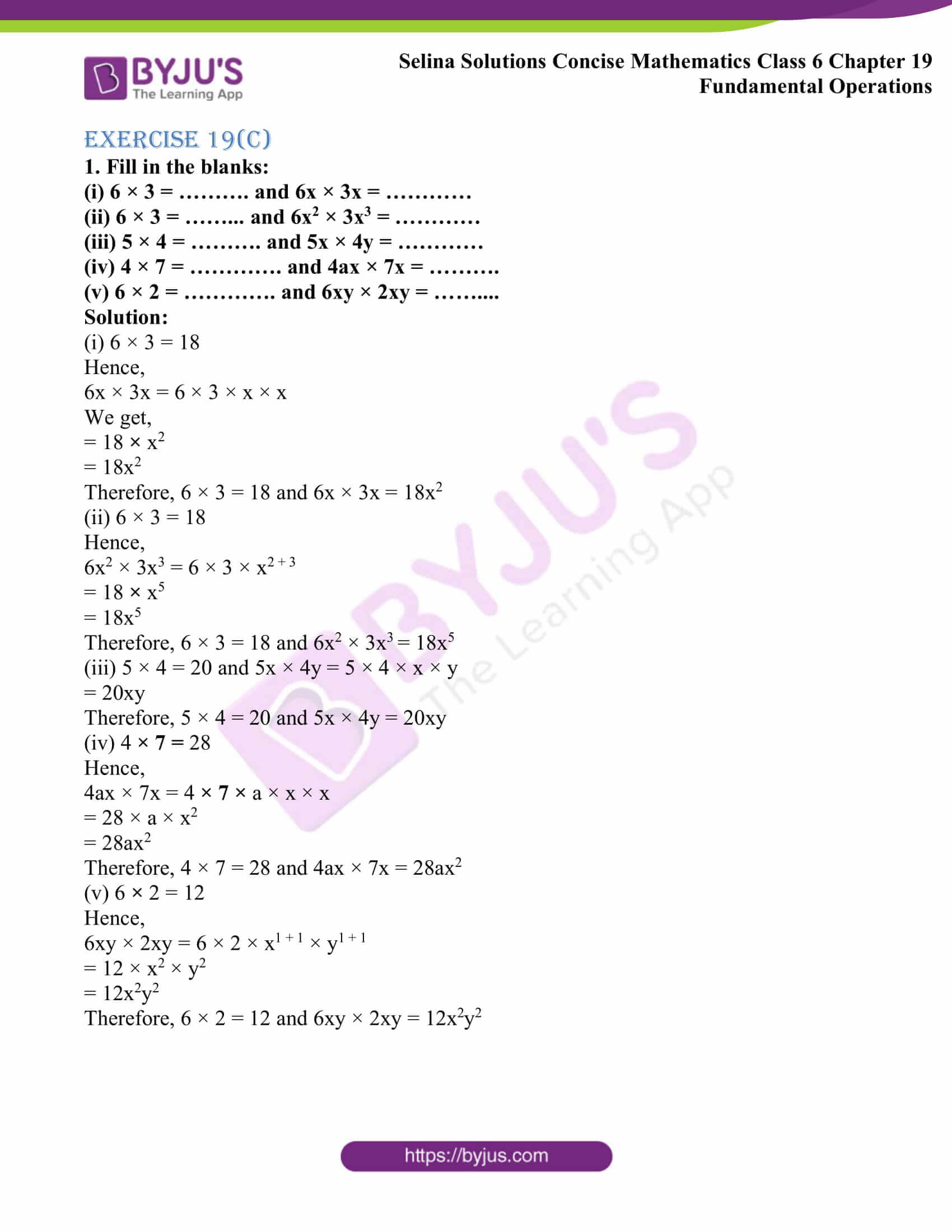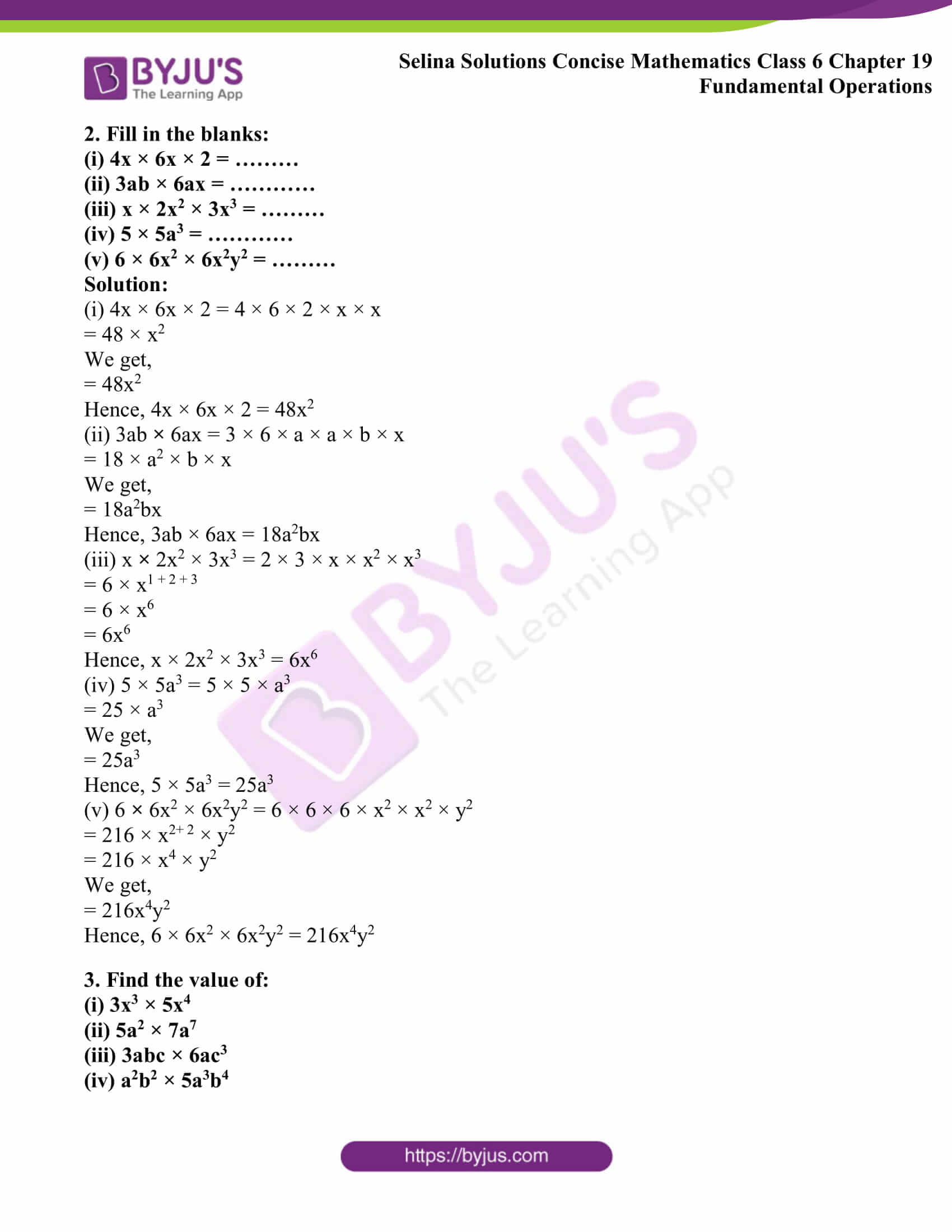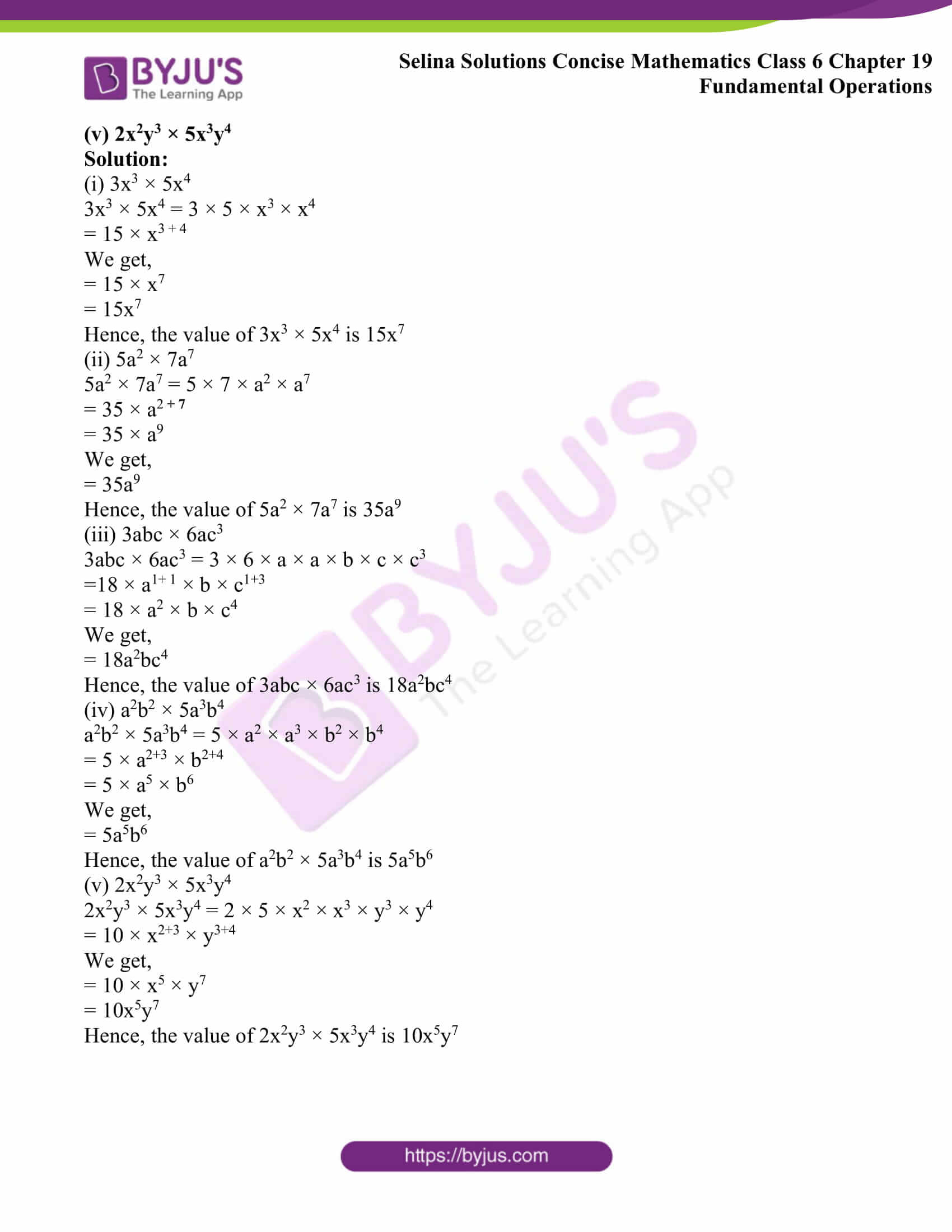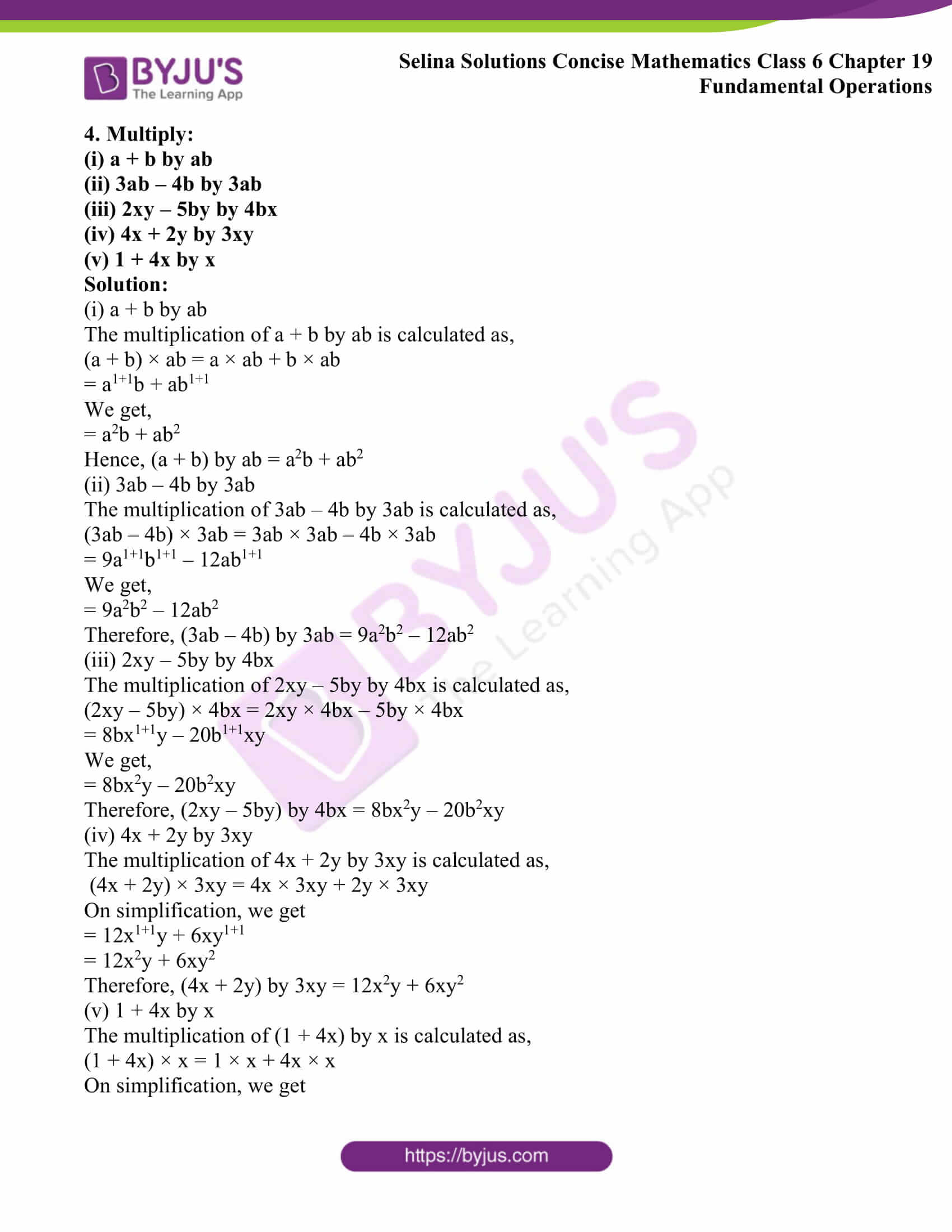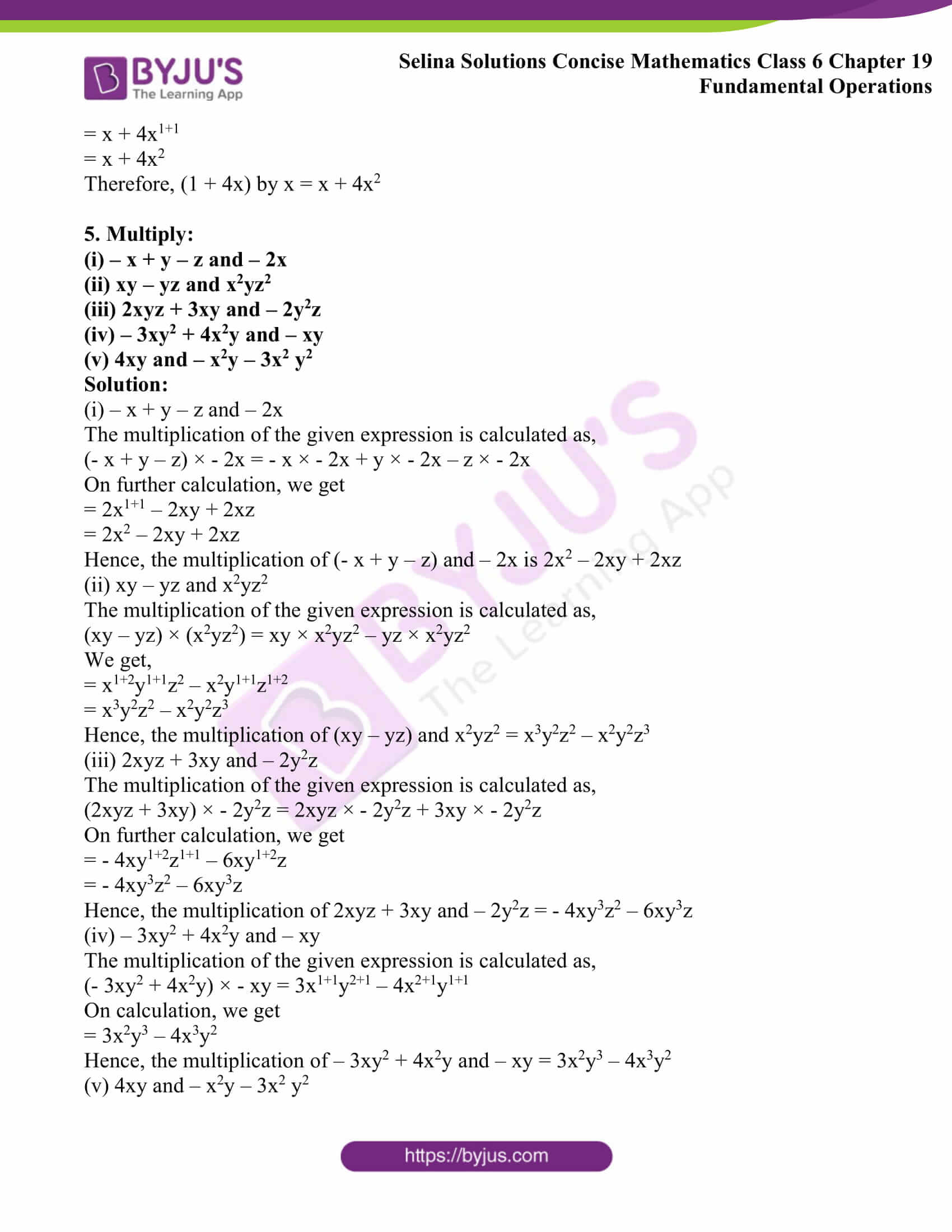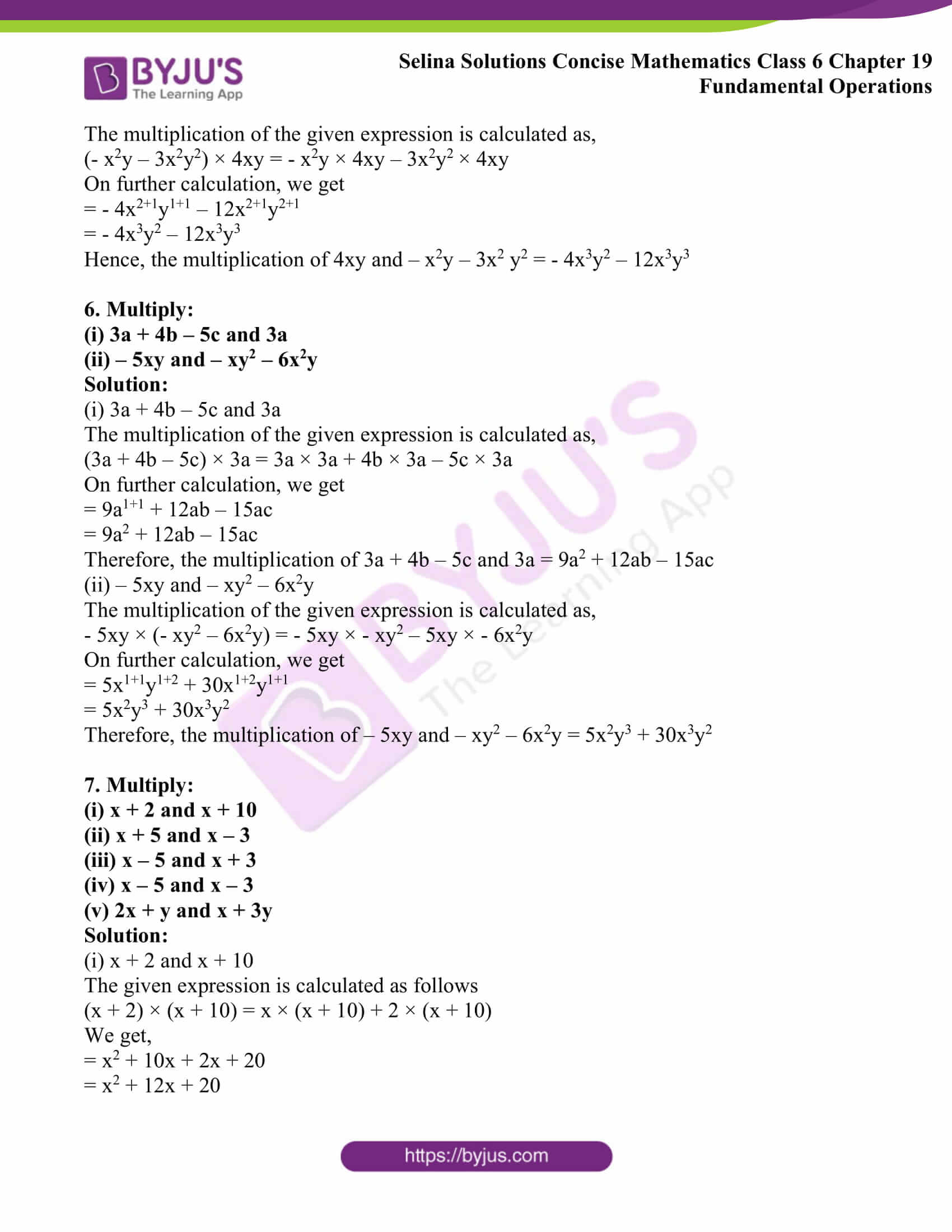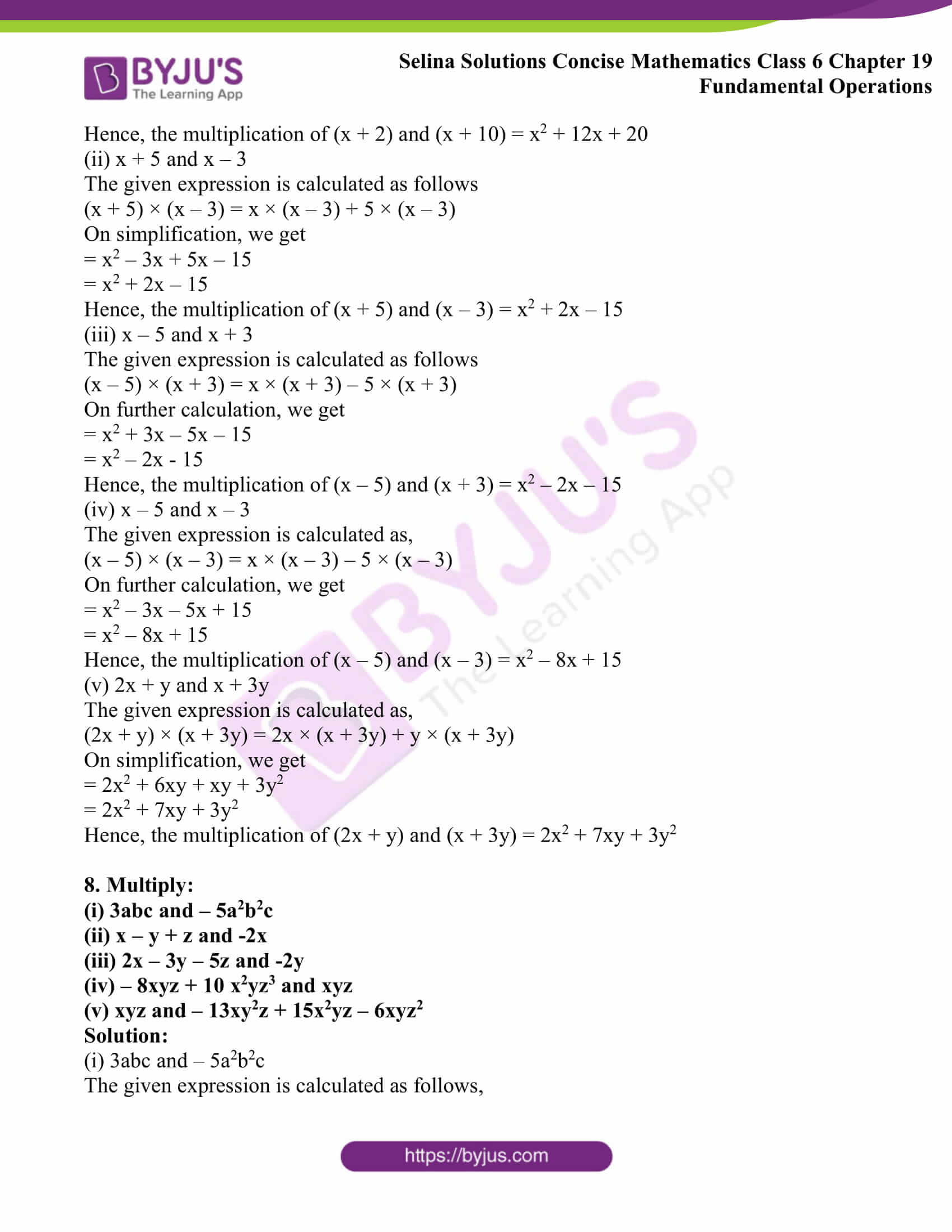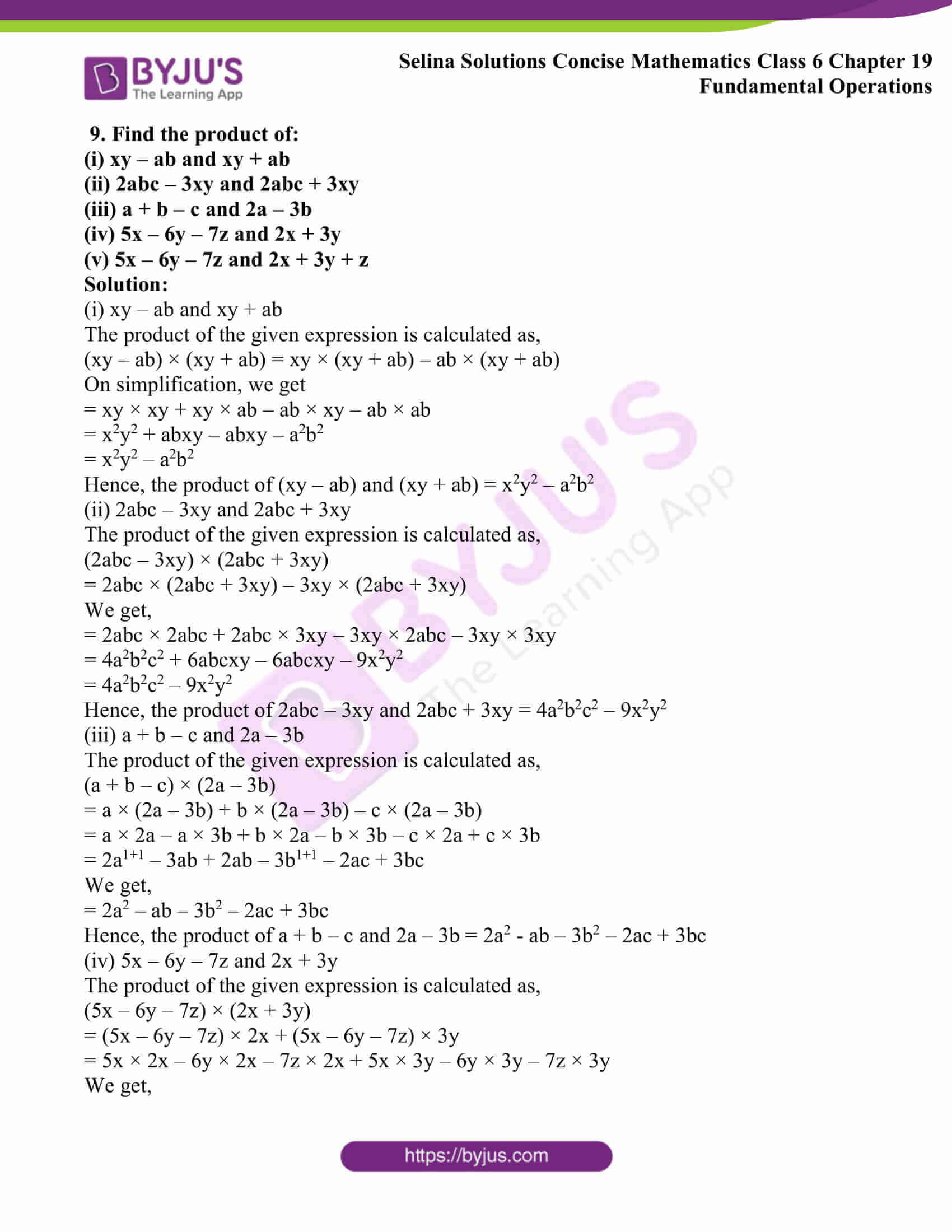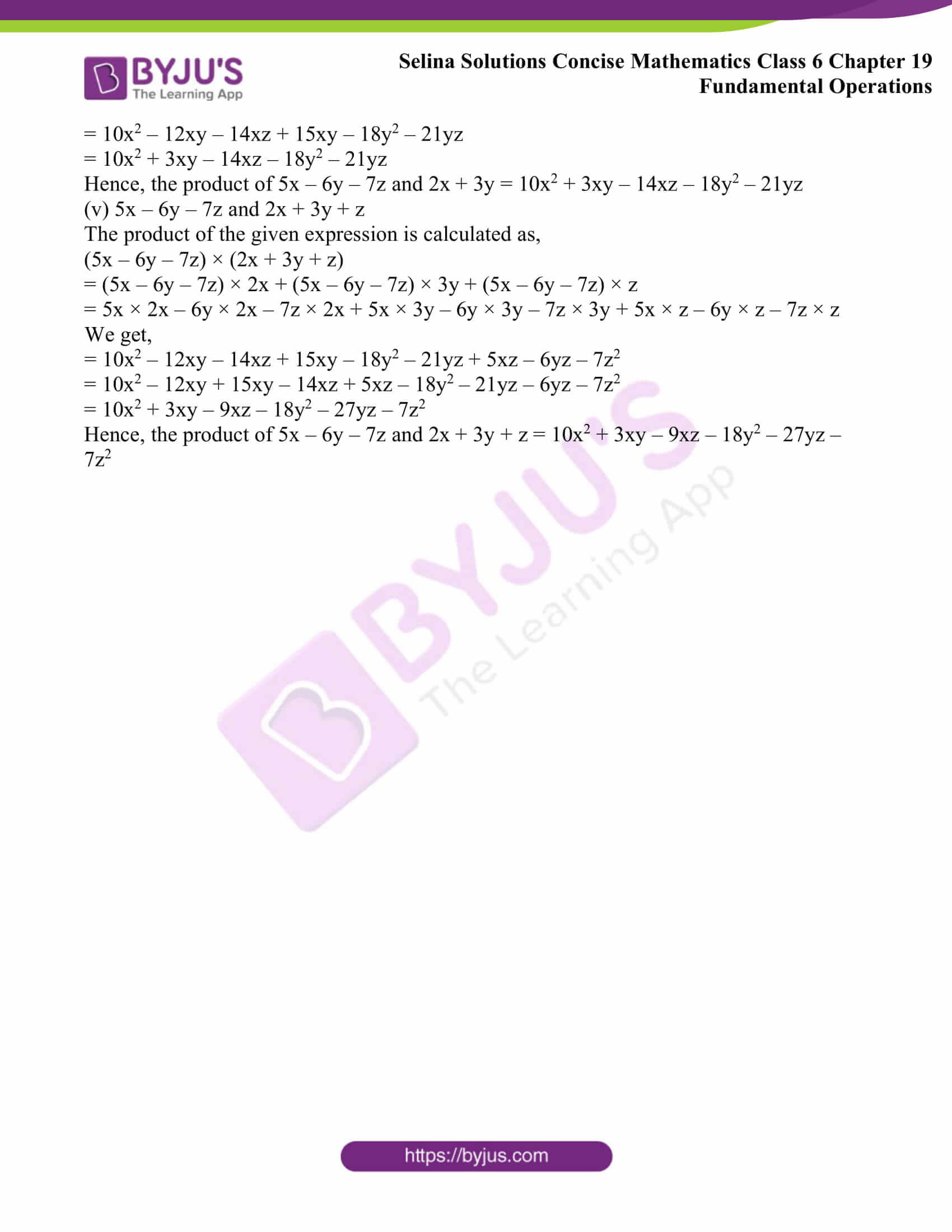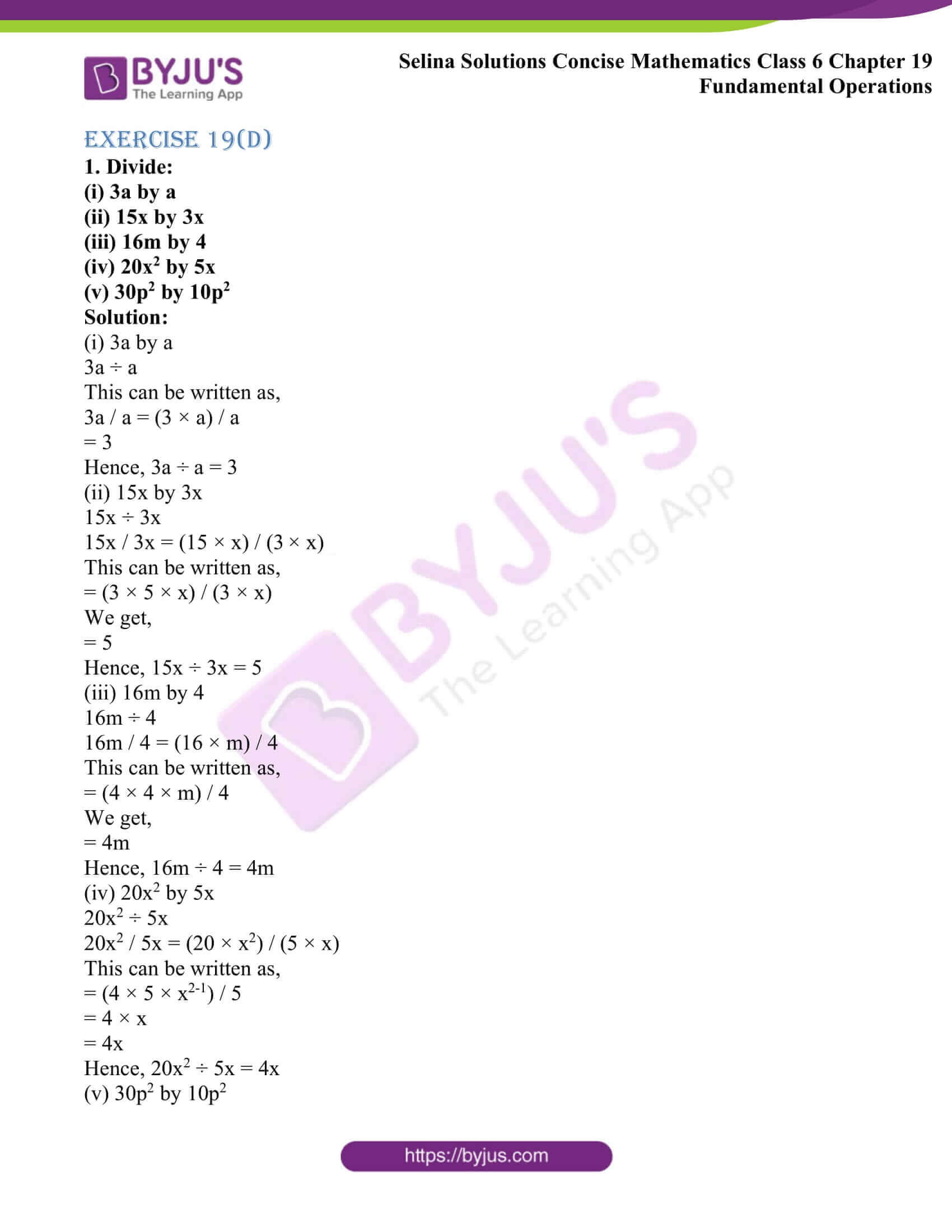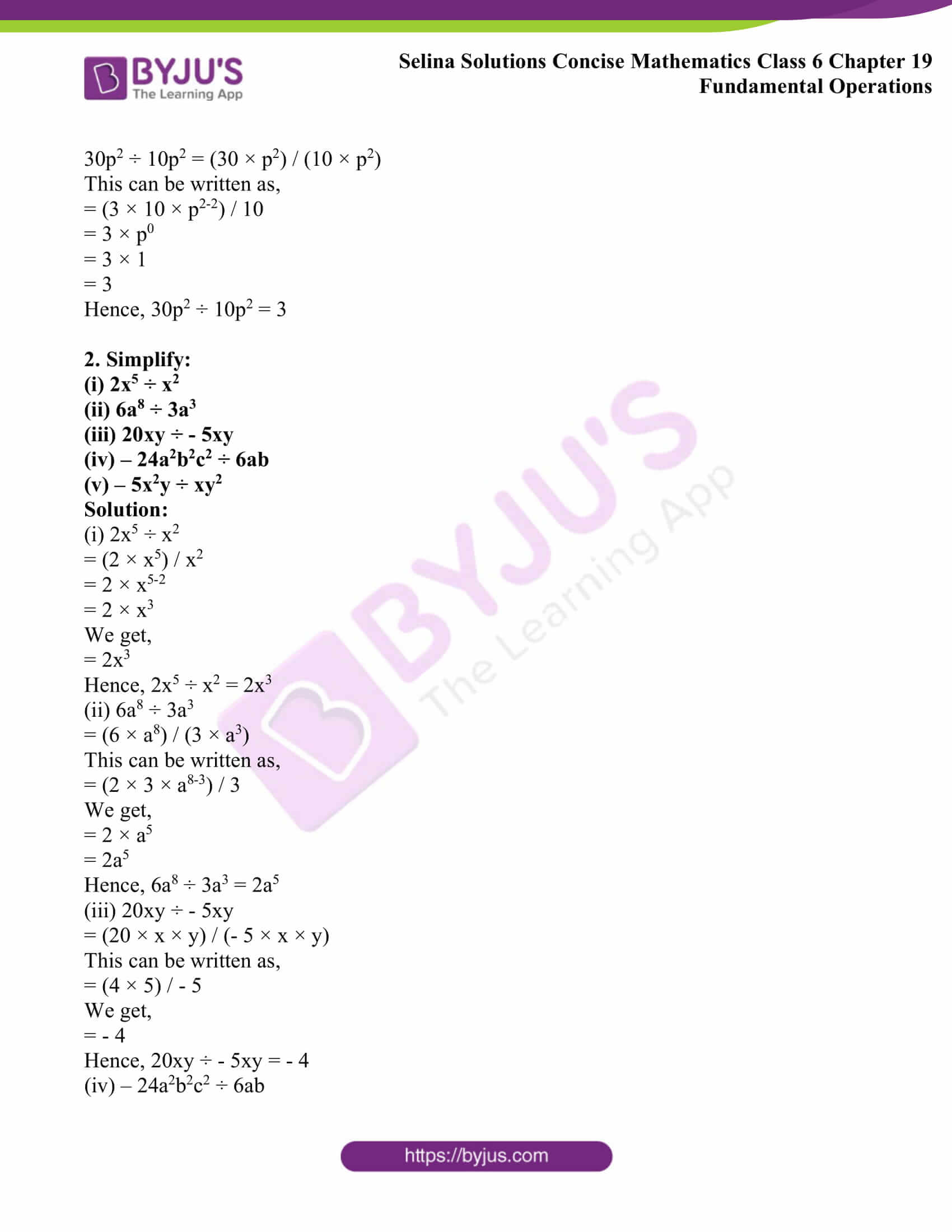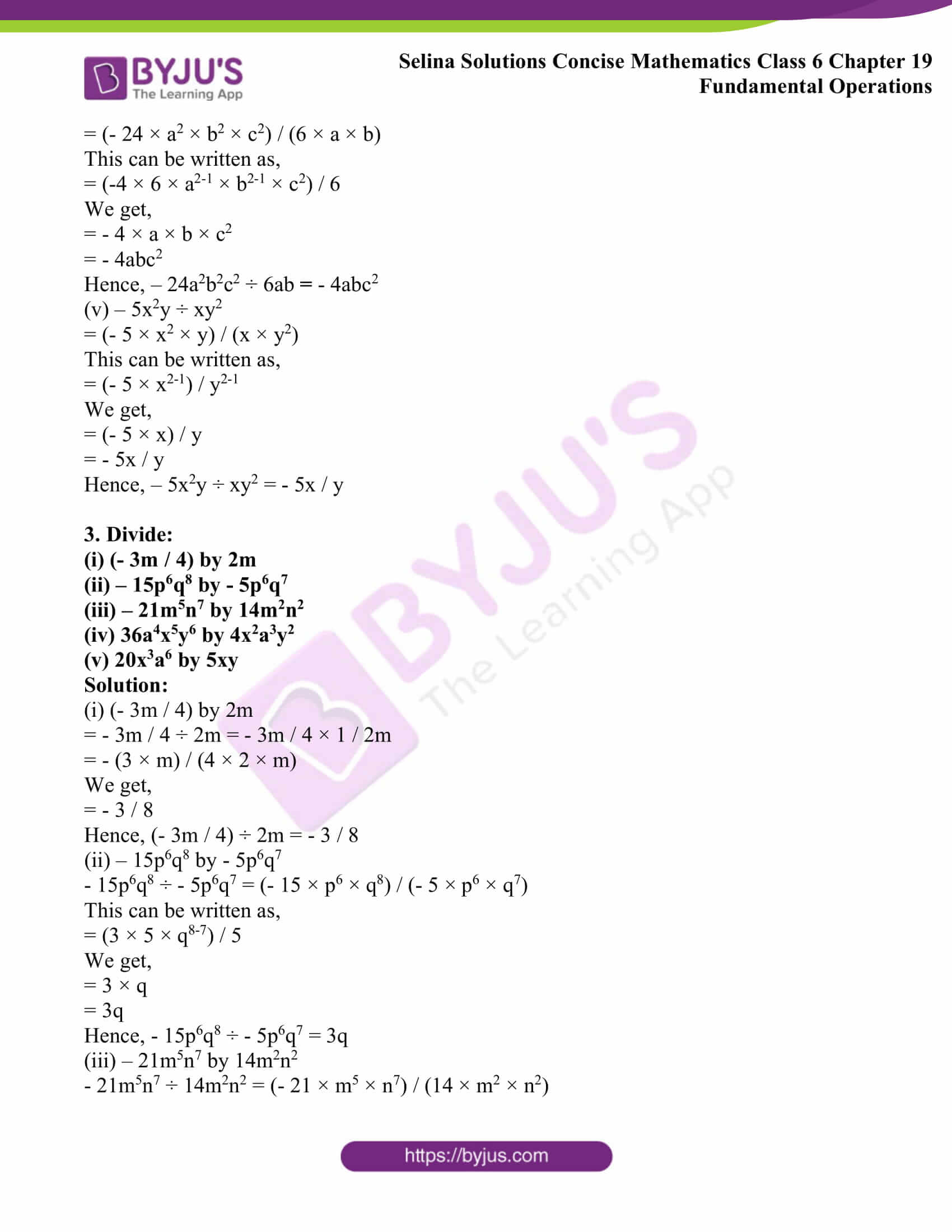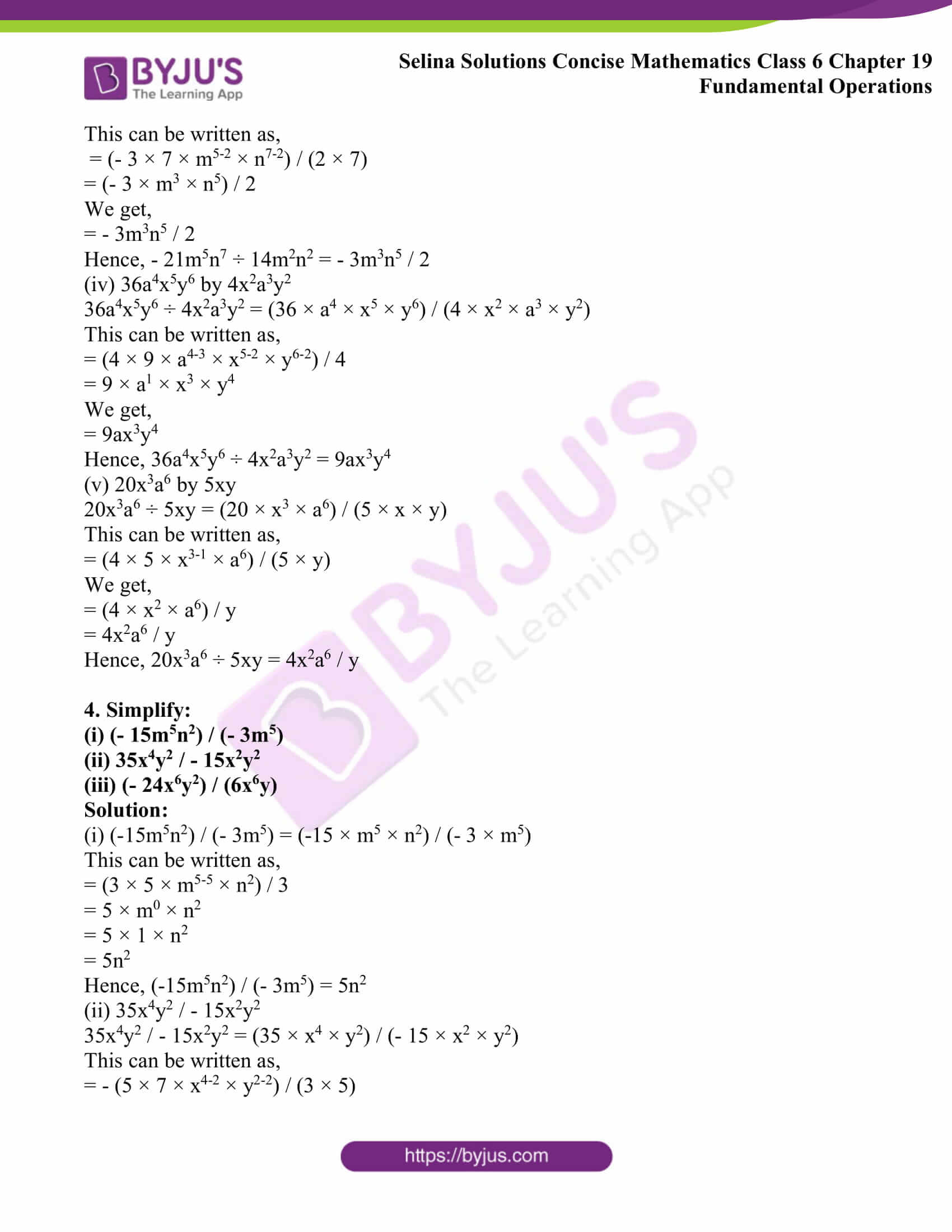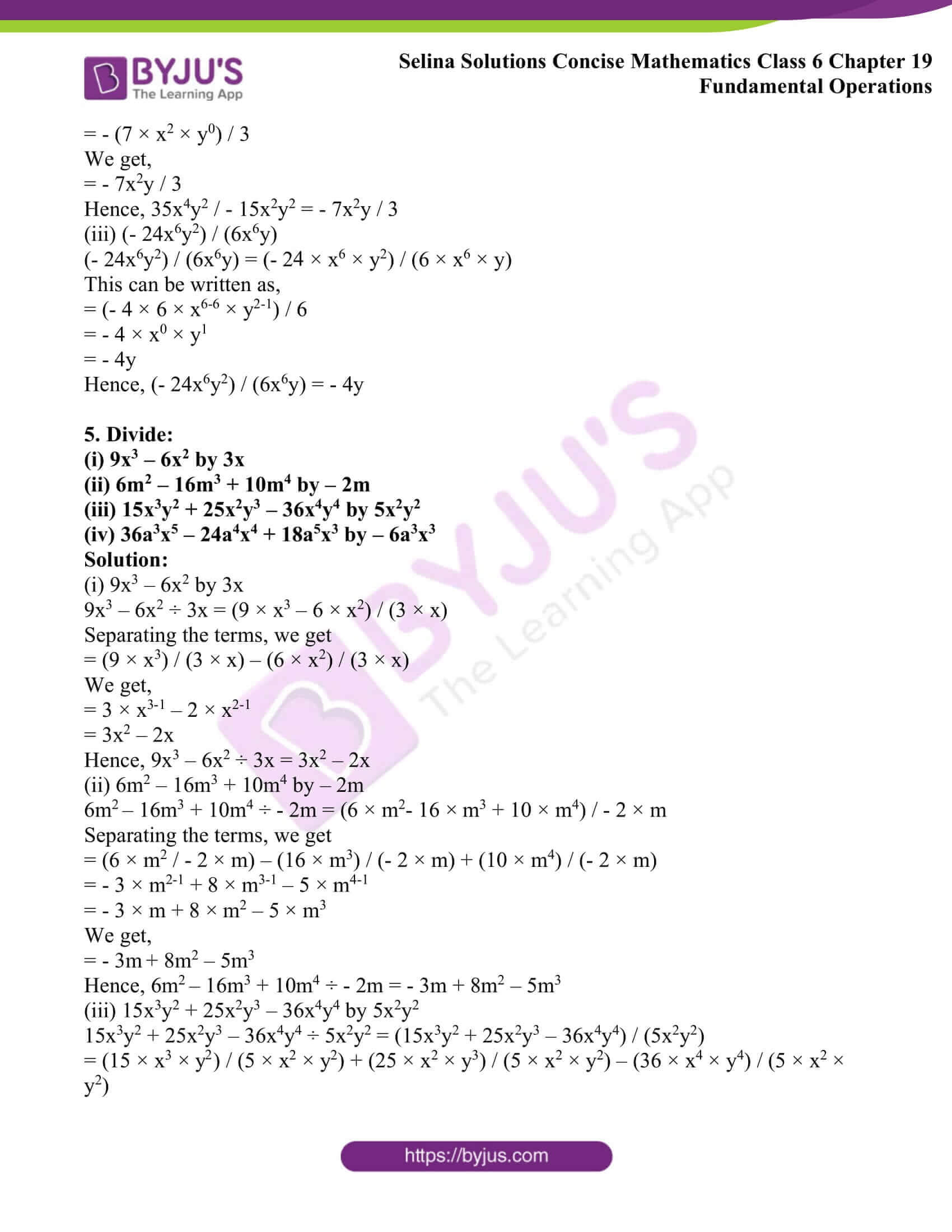### Exercises of Selina Solutions Concise Mathematics Class 6 Chapter 19: Fundamental Operations

Exercise 19(A) Solutions

Exercise 19(B) Solutions

Exercise 19(C) Solutions

Exercise 19(D) Solutions

## Access Selina Solutions Concise Mathematics Class 6 Chapter 19: Fundamental Operations

Exercise 19(A)

1. Fill in the blanks:

(i) 5 + 4 = …….. and 5x + 4x = ………

(ii) 12 + 18 = ……. and 12x2y + 18x2y = ……..

(iii) 7 + 16 = …….. and 7a + 16b = …….

(iv) 1 + 3 = ……. and x2y + 3xy2 = ……

(v) 7 – 4 = …… and 7ab – 4ab = ………

Solution:

(i) 5 + 4 = 9 and 5x + 4x = 9x

(ii) 12 + 18 = 30 and 12x2y + 18x2y = 30x2y

(iii) 7 + 16 = 23 and 7a + 16b = 7a + 16b

(iv) 1 + 3 = 4 and x2y + 3xy2 = x2y + 3xy2

(v) 7 – 4 = 3 and 7ab – 4ab = 3ab

2. Fill in the blanks:

(i) The sum of -2 and -5 = ……… and the sum of -2x and -5x = ……….

(ii) The sum of 8 and -3 = ……… and the sum of 8ab and -3ab = ………….

(iii) The sum of -15 and -4 = ……… and the sum of -15x and -4y = ………..

(iv) 15 + 8 + 3 = …….. and 15x + 8y + 3x = …………

(v) 12 – 9 + 15 = ………… and 12ab – 9ab + 15ba = ……….

Solution:

(i) The sum of -2 and -5 = – 7 and the sum of -2x and -5x = – 7x

(ii) The sum of 8 and -3 = 5 and the sum of 8ab and -3ab = 5ab

(iii) The sum of -15 and -4 = – 19 and the sum of -15x and -4y = – 15x – 4y

(iv) 15 + 8 + 3 = 26 and 15x + 8y + 3x = 18x + 8y

(v) 12 – 9 + 15 = 18 and 12ab – 9ab + 15ba = 18ab

(i) 8xy and 3xy

(ii) 2xyz, xyz and 6xyz

(iii) 2a, 3a and 4b

(iv) 3x and 2y

(v) 5m, 3n and 4p

Solution:

(i) 8xy and 3xy

The addition of 8xy and 3xy is calculated as follows

8xy + 3xy = 11xy

(ii) 2xyz, xyz and 6xyz

The addition of 2xyz, xyz and 6xyz is calculated as follows

2xyz + xyz + 6xyz = 9xyz

(iii) 2a, 3a and 4b

The addition of 2a, 3a and 4b is calculated as follows

2a + 3a + 4b = 5a + 4b

(iv) 3x and 2y

The addition of 3x and 2y is calculated as follows

3x + 2y = 3x + 2y

(v) 5m, 3n and 4p

The addition of 5m, 3n and 4p is calculated as follows

5m + 3n + 4p = 5m + 3n + 4p

4. Evaluate:

(i) 6a – a – 5a – 2a

(ii) 2b – 3b – b + 4b

(iii) 3x – 2x – 4x + 7x

(iv) 5ab + 2ab – 6ab + ab

(v) 8x – 5y – 3x + 10y

Solution:

(i) 6a – a – 5a – 2a

The value of given expression is calculated as below

6a – a – 5a – 2a = (6 – 1 – 5 – 2) a

We get,

= (5 – 5 – 2) a

= – 2a

Therefore, 6a – a – 5a – 2a = – 2a

(ii) 2b – 3b – b + 4b

The value of given expression is calculated as below

2b – 3b – b + 4b = 2b + 4b – (3 + 1) b

We get,

= 6b – 4b

= 2b

Therefore, 2b – 3b – b + 4b = 2b

(iii) 3x – 2x – 4x + 7x

The given expression is calculated as below

3x – 2x – 4x + 7x = 3x + 7x – 2x – 4x

= (3 + 7) x – (2 + 4) x

= 10x – 6x

= 4x

Therefore, 3x – 2x – 4x + 7x = 4x

(iv) 5ab + 2ab – 6ab + ab

The given expression is calculated as below

5ab + 2ab – 6ab + ab = 5ab + 2ab + ab – 6ab

We get,

= 8ab – 6ab

= 2ab

Therefore, 5ab + 2ab – 6ab + ab = 2ab

(v) 8x – 5y – 3x + 10y

The given expression is calculated as below

8x – 5y – 3x + 10y = 8x – 3x + 10y – 5y

= 5x + 5y

Therefore, 8x – 5y – 3x + 10y = 5x + 5y

5. Evaluate:

(i) -7x + 9x + 2x – 2x

(ii) 5ab – 2ab – 8ab + 6ab

(iii) -8a – 3a + 12a + 13a – 6a

(iv) 19abc – 11abc – 12abc + 14abc

Solution:

(i) -7x + 9x + 2x – 2x

The value of given expression is calculated as follows

-7x + 9x + 2x – 2x = 9x + 2x – 7x – 2x

= 11x – 9x

We get,

= 2x

Hence, -7x + 9x + 2x – 2x = 2x

(ii) 5ab – 2ab – 8ab + 6ab

The value of given expression is calculated as follows

5ab – 2ab – 8ab + 6ab = 5ab + 6ab – 2ab – 8ab

We get,

= 11ab – 10ab

= ab

Hence, 5ab – 2ab – 8ab + 6ab = ab

(iii) 8a – 3a + 12a + 13a – 6a

The value of given expression is calculated as follows

-8a – 3a + 12a + 13a – 6a = 12a + 13a – (8a + 3a + 6a)

= 25a – 17a

= 8a

Hence, -8a – 3a + 12a + 13a – 6a = 8a

(iv) 19abc – 11abc – 12abc + 14abc

The value of given expression is calculated as follows

19abc – 11abc – 12abc + 14abc = abc (19 – 11 – 12 + 14)

= abc (33 – 23)

= 10abc

Hence, 19abc – 11abc – 12abc + 14abc = 10abc

6. Subtract the first term from the second:

(i) 4ab, 6ba

(ii) 4.8b, 6.8b

(iii) 3.5abc, 10.5abc

(iv) 3(1 / 2) mn, 8(1 / 2)nm

Solution:

(i) 4ab, 6ba

The subtraction of first term from the second term is calculated as below

6ba – 4ab = 2ab

(ii) 4.8b, 6.8b

The subtraction of first term from the second term is calculated as below

6.8b – 4.8b = 2b

(iii) 3.5abc, 10.5abc

The subtraction of first term from the second term is calculated as below

10.5abc – 3.5abc = 7abc

(iv) 3(1 / 2) mn, 8(1 / 2)nm

The subtraction of first term from the second term is calculated as below

8(1 / 2)nm – 3 (1 / 2) mn = (17 / 2)nm – (7 / 2)mn

We get,

= [(17mn – 7mn) / 2]

= (10 / 2)mn

= 5mn

7. Simplify:

(i) 2a2b2 + 5ab2 + 8a2b2 – 3ab2

(ii) 4a + 3b – 2a – b

(iii) 2xy + 4yz + 5xy + 3yz – 6xy

(iv) ab + 15ab – 11ab – 2ab

(v) 6a2 – 3b2 + 2a2 + 5b2 – 4a2

Solution:

(i) 2a2b2 + 5ab2 + 8a2b2 – 3ab2

The simplified form of the given expression is calculated as follows

2a2b2 + 5ab2 + 8a2b2 – 3ab2 = 2a2b2 + 8a2b2 + 5ab2 – 3ab2

We get,

= 10a2b2 + 2ab2

Therefore, 2a2b2 + 5ab2 + 8a2b2 – 3ab2 = 10a2b2 + 2ab2

(ii) 4a + 3b – 2a – b

The simplified form of the given expression is calculated as follows

4a + 3b – 2a – b = 4a – 2a + 3b – b

= 2a + 2b

Therefore, 4a + 3b – 2a – b = 2a + 2b

(iii) 2xy + 4yz + 5xy + 3yz – 6xy

The simplified form of the given expression is calculated as follows

2xy + 4yz + 5xy + 3yz – 6xy = 2xy + 5xy – 6xy + 4yz + 3yz

= xy + 7yz

Therefore, 2xy + 4yz + 5xy + 3yz – 6xy = xy + 7yz

(iv) ab + 15ab – 11ab – 2ab

The simplified form of the given expression is calculated as follows

ab + 15ab – 11ab – 2ab = 16ab – 13ab

= 3ab

Therefore, ab + 15ab – 11ab – 2ab = 3ab

(v) 6a2 – 3b2 + 2a2 + 5b2 – 4a2

The simplified form of the given expression is calculated as follows

6a2 – 3b2 + 2a2 + 5b2 – 4a2 = 6a2 + 2a2 – 4a2 + 5b2 – 3b2

We get,

= 4a2 + 2b2

Therefore, 6a2 – 3b2 + 2a2 + 5b2 – 4a2 = 4a2 + 2b2

Exercise 19(B)

1. Find the sum of:

(i) 3a + 4b + 7c, – 5a + 3b – 6c

and 4a – 2b – 4c

(ii) 2x2 + xy – y2, – x2 + 2xy + 3y2

and 3x2 – 10xy + 4y2

(iii) x2 – x + 1, -5x2 + 2x – 2

and 3x2 – 3x + 1

(iv) a2 – ab + bc, 2ab + bc – 2a2

and – 3bc + 3a2 + ab

(v) 4x2 + 7 – 3x, 4x – x2 + 8

and – 10 + 5x – 2x2

Solution:

(i) 3a + 4b + 7c, – 5a + 3b – 6c

and 4a – 2b – 4c

The sum of 3a + 4b + 7c, – 5a + 3b – 6c and 4a – 2b – 4c is calculated as shown below

(3a + 4b + 7c) + (-5a + 3b – 6c) + (4a – 2b – 4c)

= 3a + 4a – 5a + 4b + 3b – 2b + 7c – 6c – 4c

We get,

= 2a +5b – 3c

Hence, the sum of 3a + 4b + 7c, – 5a + 3b – 6c and 4a – 2b – 4c is 3c

(ii) 2x2 + xy – y2, – x2 + 2xy + 3y2

and 3x2 – 10xy + 4y2

The sum of 2x2 + xy – y2, – x2 + 2xy + 3y2 and 3x2 – 10xy + 4y2 is calculated as shown below

(2x2 + xy – y2) + (- x2 + 2xy + 3y2) + (3x2 – 10xy + 4y2)

= 2x2 – x2 + 3x2 + xy + 2xy – 10xy + 3y2 + 4y2 – y2

We get,

= 4x2 – 7xy + 6y2

Hence, the sum of 2x2 + xy – y2, – x2 + 2xy + 3y2 and 3x2 – 10xy + 4y2 is 4x2 – 7xy + 6y2

(iii) x2 – x + 1, -5x2 + 2x – 2 and 3x2 – 3x + 1

The sum of (x2 – x + 1), (- 5x2 + 2x – 2) and (3x2 – 3x + 1) is calculated as shown below

(x2 – x + 1) + (- 5x2 + 2x – 2) + (3x2 – 3x + 1)

= x2 – 5x2 + 3x2 + 2x – x – 3x + 1 +1 – 2

= – x2 – 2x

Hence, the sum of (x2 – x + 1), (- 5x2 + 2x – 2) and (3x2 – 3x + 1) is – x2 – 2x

(iv) a2 – ab + bc, 2ab + bc – 2a2 and – 3bc + 3a2 + ab

The sum of (a2 – ab + bc), (2ab + bc – 2a2) and (- 3bc + 3a2 + ab) is calculated as shown below

(a2 – ab + bc) + (2ab + bc – 2a2) + (- 3bc + 3a2 + ab)

= a2 – 2a2 + 3a2 + 2ab + ab – ab + bc + bc – 3bc

We get,

= 2a2 +2ab – bc

Hence, the sum of (a2 – ab + bc), (2ab + bc – 2a2) and (- 3bc + 3a2 + ab) is 2a2 +2ab – bc

(v) 4x2 + 7 – 3x, 4x – x2 + 8 and – 10 + 5x – 2x2

The sum of (4x2 + 7 – 3x), (4x – x2 + 8) and (- 10 + 5x – 2x2) is calculated as shown below

(4x2 + 7 – 3x) + (4x – x2 + 8) + (- 10 + 5x – 2x2)

= 4x2 – x2 – 2x2 + 7 + 8 – 10 + 4x + 5x – 3x

We get,

= x2 + 5 + 6x

Hence, the sum of (4x2 + 7 – 3x), (4x – x2 + 8) and (- 10 + 5x – 2x2) is x2 + 5 + 6x

(i) – 17x2 – 2xy + 23y2, – 9y2 + 15x2 + 7xy

and 13x2 + 3y2 – 4xy

(ii) – x2 – 3xy + 3y2 + 8, 3x2 – 5y2 – 3 + 4xy

and – 6xy + 2x2 – 2 + y2

(iii) a3 – 2b3 + a, b3 – 2a3 + b

and – 2b + 2b3 – 5a + 4a3

Solution:

(i) The sum of (– 17x2 – 2xy + 23y2), (- 9y2 + 15x2 + 7xy) and (13x2 + 3y2 – 4xy) is calculated as follows

(– 17x2 – 2xy + 23y2) + (- 9y2 + 15x2 + 7xy) + (13x2 + 3y2 – 4xy)

= – 17x2 + 15x2 + 13x2 – 2xy – 4xy + 7xy + 23y2 + 3y2 – 9y2

We get,

= 11x2 + xy + 17y2

Therefore, the sum of (– 17x2 – 2xy + 23y2), (- 9y2 + 15x2 + 7xy) and (13x2 + 3y2 – 4xy) is 11x2 + xy + 17y2

(ii) – x2 – 3xy + 3y2 + 8, 3x2 – 5y2 – 3 + 4xy and – 6xy + 2x2 – 2 + y2

The sum of (– x2 – 3xy + 3y2 + 8), (3x2 – 5y2 – 3 + 4xy) and (– 6xy + 2x2 – 2 + y2) is calculated as follows

(– x2 – 3xy + 3y2 + 8) + (3x2 – 5y2 – 3 + 4xy) + (– 6xy + 2x2 – 2 + y2)

= – x2 + 3x2 + 2x2 – 3xy – 6xy + 4xy + 3y2 + y2 – 5y2 + 8 – 3 – 2

We get,

= 4x2 – 5xy – y2 + 3

Therefore, the sum of (– x2 – 3xy + 3y2 + 8), (3x2 – 5y2 – 3 + 4xy) and (– 6xy + 2x2 – 2 + y2) is 4x2 – 5xy – y2 + 3

(iii) a3 – 2b3 + a, b3 – 2a3 + b and – 2b + 2b3 – 5a + 4a3

The sum of (a3 – 2b3 + a), (b3 – 2a3 + b) and (– 2b + 2b3 – 5a + 4a3) is calculated as follows

(a3 – 2b3 + a) + (b3 – 2a3 + b) + (– 2b + 2b3 – 5a + 4a3)

= a3 + 4a3 – 2a3 – 2b3 + b3 + 2b3 + a – 5a + b – 2b

We get,

= 3a3 + b3 – 4a – b

Therefore, the sum of (a3 – 2b3 + a), (b3 – 2a3 + b) and (– 2b + 2b3 – 5a + 4a3) is 3a3 + b3 – 4a – b

3. Evaluate:

(i) 3a – (a + 2b)

(ii) (5x – 3y) – (x + y)

(iii) (8a + 15b) – (3b – 7a)

(iv) (8x + 7y) – (4y – 3x)

(v) 7 – (4a – 5)

Solution:

(i) 3a – (a + 2b)

The value of the given expression is calculated as below

3a – (a + 2b)

= 3a – a – 2b

= 2a – 2b

Taking 2 as common, we get

= 2(a – b)

(ii) (5x – 3y) – (x + y)

The value of the given expression is calculated as below

(5x – 3y) – (x + y)

= 5x – x – 3y – y

= 4x – 4y

Taking 4 as common, we get

= 4(x – y)

(iii) (8a + 15b) – (3b – 7a)

The value of the given expression is calculated as below

(8a + 15b) – (3b – 7a)

= 8a + 7a + 15b – 3b

On calculation, we get

= 15a + 12b

(iv) (8x + 7y) – (4y – 3x)

The value of the given expression is calculated as below

(8x + 7y) – (4y – 3x)

= 8x + 3x + 7y – 4y

On further calculation, we get

= 11x + 3y

(v) 7 – (4a – 5)

The value of the given expression is calculated as below

7 – (4a – 5)

= 7 – 4a + 5

We get,

= 12 – 4a

4. Subtract:

(i) 5a – 3b + 2c from a – 4b – 2c

(ii) 4x – 6y + 3z from 12x + 7y – 21z

(iii) 5 – a – 4b + 4c from 5a – 7b + 2c

(iv) – 8x – 12y + 17z from x – y – z

(v) 2ab + cd – ac – 2bd from ab – 2cd + 2ac + bd

Solution:

(i) 5a – 3b + 2c from a – 4b – 2c

The value of the subtraction is calculated as follows

(a – 4b – 2c) – (5a – 3b + 2c)

= a – 5a – 4b + 3b – 2c – 2c

We get,

= – 4a – b – 4c

(ii) 4x – 6y + 3z from 12x + 7y – 21z

The value of the subtraction is calculated as follows

(12x + 7y – 21z) – (4x – 6y + 3z)

= 12x – 4x + 7y + 6y – 21z – 3z

On further calculation, we get

= 8x + 13y – 24z

(iii) 5 – a – 4b + 4c from 5a – 7b + 2c

The value of the subtraction is calculated as follows

(5a – 7b + 2c) – (5 – a – 4b + 4c)

= 5a + a – 7b + 4b + 2c – 4c – 5

We get,

=6a – 3b – 2c – 5

(iv) – 8x – 12y + 17z from x – y – z

The value of the subtraction is calculated as follows

(x – y – z) – (- 8x – 12y + 17z)

= x + 8x + 12y – y – z – 17z

We get,

= 9x + 11y – 18z

(v) 2ab + cd – ac – 2bd from ab – 2cd + 2ac + bd

The value of the subtraction is calculated as follows

(ab – 2cd + 2ac + bd) – (2ab + cd – ac – 2bd)

= ab – 2ab – 2cd – cd + 2ac + ac + bd + 2bd

On calculating further, we get

= – ab – 3cd + 3ac + 3bd

5.

(i) Take – ab + bc – ca from bc – ca + ab.

(ii) Take 5x + 6y – 3z from 3x + 5y – 4z.

(iii) Take (-3 / 2) p + q – r from (1 / 2)p – (1 / 3)q – (3 / 2) r

(iv) Take 1 – a + a2 from a2 + a + 1

Solution:

(i) The value of the subtraction is calculated as,

(bc – ca + ab) – (-ab + bc – ca)

= bc – bc – ca + ca + ab + ab

We get,

= 2ab

Hence, (bc – ca + ab) – (-ab + bc – ca) = 2ab

(ii) The value of the subtraction is calculated as,

(3x + 5y – 4z) – (5x + 6y – 3z)

= 3x – 5x + 5y – 6y – 4z + 3z

On simplification, we get

= – 2x – y – z

Hence, (3x + 5y – 4z) – (5x + 6y – 3z) = – 2x – y – z

(iii) The value of the subtraction is calculated as,

[(1 / 2)p – (1 / 3)q – (3 / 2) r] – [(-3 / 2) p + q – r]

= (1 / 2)p + (3 / 2)p – (1 / 3)q – q – (3 / 2)r + r

On further calculation, we get

= 2p – (4 / 3)q – (1 / 2)r

Hence, [(1 / 2)p – (1 / 3)q – (3 / 2) r] – [(-3 / 2) p + q – r] = 2p – (4 / 3)q – (1 / 2)r

(iv) The value of the subtraction is calculated as,

(a2 + a + 1) – (1 – a + a2)

= a2 – a2 + a + a + 1 – 1

We get,

= a + a

= 2a

6. From the sum of x + y – 2z and 2x – y + z subtract x + y + z.

Solution:

The value of terms as per the question is calculated as follows

(x + y – 2z) + (2x – y + z) – (x + y + z)

= x + 2x – x + y – y – y – 2z – z + z

We get,

= 2x – y – 2z

Therefore, (x + y – 2z) + (2x – y + z) – (x + y + z) = 2x – y – 2z

7. From the sum of 3a – 2b + 4c and 3b – 2c subtract a – b – c.

Solution:

The value of terms as per the question is calculated as shown below

(3a – 2b + 4c) + (3b – 2c) – (a – b – c)

= 3a – 2b + 4c + 3b – 2c – a + b + c

On further calculation, we get

= 3a – a + 3b + b – 2b + 4c + c – 2c

= 2a + 2b + 3c

Hence, (3a – 2b + 4c) + (3b – 2c) – (a – b – c) = 2a + 2b + 3c

8. Subtract x – 2y – z from the sum of 3x – y + z and x + y – 3z.

Solution:

The value of terms as per the question is calculated as follows

(3x – y + z) + (x + y – 3z) – (x – 2y – z)

= 3x + x – x – y + y + 2y + z – 3z + z

We get,

= 3x + 2y – z

Therefore, (3x – y + z) + (x + y – 3z) – (x – 2y – z) = 3x + 2y – z

9. Subtract the sum of x + y and x – z from the sum of x – 2z and x + y + z

Solution:

The value of terms as per the question is calculated as follows

(x – 2z) + (x + y + z) – {(x + y) + (x – z)}

On further calculation, we get

= x + x – x –x + y – y + z + z – 2z

We get,

= 0

Therefore, (x – 2z) + (x + y + z) – {(x + y) + (x – z)} = 0

10. By how much should x + 2y – 3z be increased to get 3x?

Solution:

The terms calculated as per the question is as follows

3x – (x + 2y – 3z)

= 3x – x – 2y + 3z

We get,

= 2x – 2y + 3z

11. The sum of two expressions is 5x2 – 3y2. If one of them is 3x2 + 4xy – y2, find the other.

Solution:

The other expression is calculated as follows

(5x2 – 3y2) – (3x2 + 4xy – y2)

= 5x2 – 3x2 – 4xy – 3y2 + y2

We get,

= 2x2 – 4xy – 2y2

12. The sum of two expressions is 3a2 + 2ab – b2. If one of them is 2a2 + 3b2, find the other.

Solution:

The other expression is calculated as follows

(3a2 + 2ab – b2) – (2a2 + 3b2)

On simplification, we get

=3a2 – 2a2 – b2 – 3b2 + 2ab

= a2– 4b2 + 2ab

Exercise 19(C)

1. Fill in the blanks:

(i) 6 × 3 = ………. and 6x × 3x = …………

(ii) 6 × 3 = ……… and 6x2 × 3x3 = …………

(iii) 5 × 4 = ………. and 5x × 4y = …………

(iv) 4 × 7 = …………. and 4ax × 7x = ……….

(v) 6 × 2 = …………. and 6xy × 2xy = ……….

Solution:

(i) 6 × 3 = 18

Hence,

6x × 3x = 6 × 3 × x × x

We get,

= 18 × x2

= 18x2

Therefore, 6 × 3 = 18 and 6x × 3x = 18x2

(ii) 6 × 3 = 18

Hence,

6x2 × 3x3 = 6 × 3 × x2 + 3

= 18 × x5

= 18x5

Therefore, 6 × 3 = 18 and 6x2 × 3x3 = 18x5

(iii) 5 × 4 = 20 and 5x × 4y = 5 × 4 × x × y

= 20xy

Therefore, 5 × 4 = 20 and 5x × 4y = 20xy

(iv) 4 × 7 = 28

Hence,

4ax × 7x = 4 × 7 × a × x × x

= 28 × a × x2

= 28ax2

Therefore, 4 × 7 = 28 and 4ax × 7x = 28ax2

(v) 6 × 2 = 12

Hence,

6xy × 2xy = 6 × 2 × x1 + 1 × y1 + 1

= 12 × x2 × y2

= 12x2y2

Therefore, 6 × 2 = 12 and 6xy × 2xy = 12x2y2

2. Fill in the blanks:

(i) 4x × 6x × 2 = ………

(ii) 3ab × 6ax = …………

(iii) x × 2x2 × 3x3 = ………

(iv) 5 × 5a3 = …………

(v) 6 × 6x2 × 6x2y2 = ………

Solution:

(i) 4x × 6x × 2 = 4 × 6 × 2 × x × x

= 48 × x2

We get,

= 48x2

Hence, 4x × 6x × 2 = 48x2

(ii) 3ab × 6ax = 3 × 6 × a × a × b × x

= 18 × a2 × b × x

We get,

= 18a2bx

Hence, 3ab × 6ax = 18a2bx

(iii) x × 2x2 × 3x3 = 2 × 3 × x × x2 × x3

= 6 × x1 + 2 + 3

= 6 × x6

= 6x6

Hence, x × 2x2 × 3x3 = 6x6

(iv) 5 × 5a3 = 5 × 5 × a3

= 25 × a3

We get,

= 25a3

Hence, 5 × 5a3 = 25a3

(v) 6 × 6x2 × 6x2y2 = 6 × 6 × 6 × x2 × x2 × y2

= 216 × x2+ 2 × y2

= 216 × x4 × y2

We get,

= 216x4y2

Hence, 6 × 6x2 × 6x2y2 = 216x4y2

3. Find the value of:

(i) 3x3 × 5x4

(ii) 5a2 × 7a7

(iii) 3abc × 6ac3

(iv) a2b2 × 5a3b4

(v) 2x2y3 × 5x3y4

Solution:

(i) 3x3 × 5x4

3x3 × 5x4 = 3 × 5 × x3 × x4

= 15 × x3 + 4

We get,

= 15 × x7

= 15x7

Hence, the value of 3x3 × 5x4 is 15x7

(ii) 5a2 × 7a7

5a2 × 7a7 = 5 × 7 × a2 × a7

= 35 × a2 + 7

= 35 × a9

We get,

= 35a9

Hence, the value of 5a2 × 7a7 is 35a9

(iii) 3abc × 6ac3

3abc × 6ac3 = 3 × 6 × a × a × b × c × c3

=18 × a1+ 1 × b × c1+3

= 18 × a2 × b × c4

We get,

= 18a2bc4

Hence, the value of 3abc × 6ac3 is 18a2bc4

(iv) a2b2 × 5a3b4

a2b2 × 5a3b4 = 5 × a2 × a3 × b2 × b4

= 5 × a2+3 × b2+4

= 5 × a5 × b6

We get,

= 5a5b6

Hence, the value of a2b2 × 5a3b4 is 5a5b6

(v) 2x2y3 × 5x3y4

2x2y3 × 5x3y4 = 2 × 5 × x2 × x3 × y3 × y4

= 10 × x2+3 × y3+4

We get,

= 10 × x5 × y7

= 10x5y7

Hence, the value of 2x2y3 × 5x3y4 is 10x5y7

4. Multiply:

(i) a + b by ab

(ii) 3ab – 4b by 3ab

(iii) 2xy – 5by by 4bx

(iv) 4x + 2y by 3xy

(v) 1 + 4x by x

Solution:

(i) a + b by ab

The multiplication of a + b by ab is calculated as,

(a + b) × ab = a × ab + b × ab

= a1+1b + ab1+1

We get,

= a2b + ab2

Hence, (a + b) by ab = a2b + ab2

(ii) 3ab – 4b by 3ab

The multiplication of 3ab – 4b by 3ab is calculated as,

(3ab – 4b) × 3ab = 3ab × 3ab – 4b × 3ab

= 9a1+1b1+1 – 12ab1+1

We get,

= 9a2b2 – 12ab2

Therefore, (3ab – 4b) by 3ab = 9a2b2 – 12ab2

(iii) 2xy – 5by by 4bx

The multiplication of 2xy – 5by by 4bx is calculated as,

(2xy – 5by) × 4bx = 2xy × 4bx – 5by × 4bx

= 8bx1+1y – 20b1+1xy

We get,

= 8bx2y – 20b2xy

Therefore, (2xy – 5by) by 4bx = 8bx2y – 20b2xy

(iv) 4x + 2y by 3xy

The multiplication of 4x + 2y by 3xy is calculated as,

(4x + 2y) × 3xy = 4x × 3xy + 2y × 3xy

On simplification, we get

= 12x1+1y + 6xy1+1

= 12x2y + 6xy2

Therefore, (4x + 2y) by 3xy = 12x2y + 6xy2

(v) 1 + 4x by x

The multiplication of (1 + 4x) by x is calculated as,

(1 + 4x) × x = 1 × x + 4x × x

On simplification, we get

= x + 4x1+1

= x + 4x2

Therefore, (1 + 4x) by x = x + 4x2

5. Multiply:

(i) – x + y – z and – 2x

(ii) xy – yz and x2yz2

(iii) 2xyz + 3xy and – 2y2z

(iv) – 3xy2 + 4x2y and – xy

(v) 4xy and – x2y – 3x2 y2

Solution:

(i) – x + y – z and – 2x

The multiplication of the given expression is calculated as,

(- x + y – z) × – 2x = – x × – 2x + y × – 2x – z × – 2x

On further calculation, we get

= 2x1+1 – 2xy + 2xz

= 2x2 – 2xy + 2xz

Hence, the multiplication of (- x + y – z) and – 2x is 2x2 – 2xy + 2xz

(ii) xy – yz and x2yz2

The multiplication of the given expression is calculated as,

(xy – yz) × (x2yz2) = xy × x2yz2 – yz × x2yz2

We get,

= x1+2y1+1z2 – x2y1+1z1+2

= x3y2z2 – x2y2z3

Hence, the multiplication of (xy – yz) and x2yz2 = x3y2z2 – x2y2z3

(iii) 2xyz + 3xy and – 2y2z

The multiplication of the given expression is calculated as,

(2xyz + 3xy) × – 2y2z = 2xyz × – 2y2z + 3xy × – 2y2z

On further calculation, we get

= – 4xy1+2z1+1 – 6xy1+2z

= – 4xy3z2 – 6xy3z

Hence, the multiplication of 2xyz + 3xy and – 2y2z = – 4xy3z2 – 6xy3z

(iv) – 3xy2 + 4x2y and – xy

The multiplication of the given expression is calculated as,

(- 3xy2 + 4x2y) × – xy = 3x1+1y2+1 – 4x2+1y1+1

On calculation, we get

= 3x2y3 – 4x3y2

Hence, the multiplication of – 3xy2 + 4x2y and – xy = 3x2y3 – 4x3y2

(v) 4xy and – x2y – 3x2 y2

The multiplication of the given expression is calculated as,

(- x2y – 3x2y2) × 4xy = – x2y × 4xy – 3x2y2 × 4xy

On further calculation, we get

= – 4x2+1y1+1 – 12x2+1y2+1

= – 4x3y2 – 12x3y3

Hence, the multiplication of 4xy and – x2y – 3x2 y2 = – 4x3y2 – 12x3y3

6. Multiply:

(i) 3a + 4b – 5c and 3a

(ii) – 5xy and – xy2 – 6x2y

Solution:

(i) 3a + 4b – 5c and 3a

The multiplication of the given expression is calculated as,

(3a + 4b – 5c) × 3a = 3a × 3a + 4b × 3a – 5c × 3a

On further calculation, we get

= 9a1+1 + 12ab – 15ac

= 9a2 + 12ab – 15ac

Therefore, the multiplication of 3a + 4b – 5c and 3a = 9a2 + 12ab – 15ac

(ii) – 5xy and – xy2 – 6x2y

The multiplication of the given expression is calculated as,

– 5xy × (- xy2 – 6x2y) = – 5xy × – xy2 – 5xy × – 6x2y

On further calculation, we get

= 5x1+1y1+2 + 30x1+2y1+1

= 5x2y3 + 30x3y2

Therefore, the multiplication of – 5xy and – xy2 – 6x2y = 5x2y3 + 30x3y2

7. Multiply:

(i) x + 2 and x + 10

(ii) x + 5 and x – 3

(iii) x – 5 and x + 3

(iv) x – 5 and x – 3

(v) 2x + y and x + 3y

Solution:

(i) x + 2 and x + 10

The given expression is calculated as follows

(x + 2) × (x + 10) = x × (x + 10) + 2 × (x + 10)

We get,

= x2 + 10x + 2x + 20

= x2 + 12x + 20

Hence, the multiplication of (x + 2) and (x + 10) = x2 + 12x + 20

(ii) x + 5 and x – 3

The given expression is calculated as follows

(x + 5) × (x – 3) = x × (x – 3) + 5 × (x – 3)

On simplification, we get

= x2 – 3x + 5x – 15

= x2 + 2x – 15

Hence, the multiplication of (x + 5) and (x – 3) = x2 + 2x – 15

(iii) x – 5 and x + 3

The given expression is calculated as follows

(x – 5) × (x + 3) = x × (x + 3) – 5 × (x + 3)

On further calculation, we get

= x2 + 3x – 5x – 15

= x2 – 2x – 15

Hence, the multiplication of (x – 5) and (x + 3) = x2 – 2x – 15

(iv) x – 5 and x – 3

The given expression is calculated as,

(x – 5) × (x – 3) = x × (x – 3) – 5 × (x – 3)

On further calculation, we get

= x2 – 3x – 5x + 15

= x2 – 8x + 15

Hence, the multiplication of (x – 5) and (x – 3) = x2 – 8x + 15

(v) 2x + y and x + 3y

The given expression is calculated as,

(2x + y) × (x + 3y) = 2x × (x + 3y) + y × (x + 3y)

On simplification, we get

= 2x2 + 6xy + xy + 3y2

= 2x2 + 7xy + 3y2

Hence, the multiplication of (2x + y) and (x + 3y) = 2x2 + 7xy + 3y2

8. Multiply:

(i) 3abc and – 5a2b2c

(ii) x – y + z and -2x

(iii) 2x – 3y – 5z and -2y

(iv) – 8xyz + 10 x2yz3 and xyz

(v) xyz and – 13xy2z + 15x2yz – 6xyz2

Solution:

(i) 3abc and – 5a2b2c

The given expression is calculated as follows,

3abc × – 5a2b2c = 3 × – 5 × a × a2 × b × b2 × c × c

On further calculation, we get

= – 15 × a1+2 × b1+2 × c1+1

= – 15 × a3 × b3 × c2

= – 15a3b3c2

Therefore, the multiplication of 3abc and – 5a2b2c = – 15a3b3c2

(ii) x – y + z and -2x

The given expression is calculated as follows,

(x – y + z) × – 2x = x × – 2x – y × – 2x + z × – 2x

On simplification, we get

= – 2x1+1 + 2xy – 2xz

= – 2x2 + 2xy – 2xz

Therefore, the multiplication of x – y + z and -2x = – 2x2 + 2xy – 2xz

(iii) 2x – 3y – 5z and -2y

The given expression is calculated as follows,

(2x – 3y – 5z) × – 2y = 2x × – 2y – 3y × – 2y – 5z × – 2y

On further calculation, we get

= – 4xy + 6y1+1 + 10yz

= – 4xy + 6y2 + 10yz

Therefore, the multiplication of 2x – 3y – 5z and -2y = – 4xy + 6y2 + 10yz

(iv) – 8xyz + 10 x2yz3 and xyz

The given expression is calculated as follows,

(- 8xyz + 10x2yz3) × xyz = – 8xyz × xyz + 10x2yz3 × xyz

On further calculation, we get

= – 8x1+1y1+1z1+1 + 10x2+1y1+1z3+1

= – 8x2y2z2 + 10x3y2z4

Therefore, the multiplication of – 8xyz + 10 x2yz3 and xyz = – 8x2y2z2 + 10x3y2z4

(v) xyz and – 13xy2z + 15x2yz – 6xyz2

The given expression is calculated as follows,

xyz × (- 13xy2z + 15x2yz – 6xyz2) = xyz × – 13xy2z + xyz ×15x2yz – xyz × 6xyz2

On simplification, we get

= – 13x1+1y1+2z1+1 + 15x1+2y1+1z1+1 – 6x1+1y1+1z1+2

We get,

= – 13x2y3z2 + 15x3y2z2 – 6x2y2z3

Therefore, the multiplication of xyz and – 13xy2z + 15x2yz – 6xyz2 = – 13x2y3z2 + 15x3y2z2 – 6x2y2z3

9. Find the product of:

(i) xy – ab and xy + ab

(ii) 2abc – 3xy and 2abc + 3xy

(iii) a + b – c and 2a – 3b

(iv) 5x – 6y – 7z and 2x + 3y

(v) 5x – 6y – 7z and 2x + 3y + z

Solution:

(i) xy – ab and xy + ab

The product of the given expression is calculated as,

(xy – ab) × (xy + ab) = xy × (xy + ab) – ab × (xy + ab)

On simplification, we get

= xy × xy + xy × ab – ab × xy – ab × ab

= x2y2 + abxy – abxy – a2b2

= x2y2 – a2b2

Hence, the product of (xy – ab) and (xy + ab) = x2y2 – a2b2

(ii) 2abc – 3xy and 2abc + 3xy

The product of the given expression is calculated as,

(2abc – 3xy) × (2abc + 3xy)

= 2abc × (2abc + 3xy) – 3xy × (2abc + 3xy)

We get,

= 2abc × 2abc + 2abc × 3xy – 3xy × 2abc – 3xy × 3xy

= 4a2b2c2 + 6abcxy – 6abcxy – 9x2y2

= 4a2b2c2 – 9x2y2

Hence, the product of 2abc – 3xy and 2abc + 3xy = 4a2b2c2 – 9x2y2

(iii) a + b – c and 2a – 3b

The product of the given expression is calculated as,

(a + b – c) × (2a – 3b)

= a × (2a – 3b) + b × (2a – 3b) – c × (2a – 3b)

= a × 2a – a × 3b + b × 2a – b × 3b – c × 2a + c × 3b

= 2a1+1 – 3ab + 2ab – 3b1+1 – 2ac + 3bc

We get,

= 2a2 – ab – 3b2 – 2ac + 3bc

Hence, the product of a + b – c and 2a – 3b = 2a2 – ab – 3b2 – 2ac + 3bc

(iv) 5x – 6y – 7z and 2x + 3y

The product of the given expression is calculated as,

(5x – 6y – 7z) × (2x + 3y)

= (5x – 6y – 7z) × 2x + (5x – 6y – 7z) × 3y

= 5x × 2x – 6y × 2x – 7z × 2x + 5x × 3y – 6y × 3y – 7z × 3y

We get,

= 10x2 – 12xy – 14xz + 15xy – 18y2 – 21yz

= 10x2 + 3xy – 14xz – 18y2 – 21yz

Hence, the product of 5x – 6y – 7z and 2x + 3y = 10x2 + 3xy – 14xz – 18y2 – 21yz

(v) 5x – 6y – 7z and 2x + 3y + z

The product of the given expression is calculated as,

(5x – 6y – 7z) × (2x + 3y + z)

= (5x – 6y – 7z) × 2x + (5x – 6y – 7z) × 3y + (5x – 6y – 7z) × z

= 5x × 2x – 6y × 2x – 7z × 2x + 5x × 3y – 6y × 3y – 7z × 3y + 5x × z – 6y × z – 7z × z

We get,

= 10x2 – 12xy – 14xz + 15xy – 18y2 – 21yz + 5xz – 6yz – 7z2

= 10x2 – 12xy + 15xy – 14xz + 5xz – 18y2 – 21yz – 6yz – 7z2

= 10x2 + 3xy – 9xz – 18y2 – 27yz – 7z2

Hence, the product of 5x – 6y – 7z and 2x + 3y + z = 10x2 + 3xy – 9xz – 18y2 – 27yz – 7z2

Exercise 19(D)

1. Divide:

(i) 3a by a

(ii) 15x by 3x

(iii) 16m by 4

(iv) 20x2 by 5x

(v) 30p2 by 10p2

Solution:

(i) 3a by a

3a ÷ a

This can be written as,

3a / a = (3 × a) / a

= 3

Hence, 3a ÷ a = 3

(ii) 15x by 3x

15x ÷ 3x

15x / 3x = (15 × x) / (3 × x)

This can be written as,

= (3 × 5 × x) / (3 × x)

We get,

= 5

Hence, 15x ÷ 3x = 5

(iii) 16m by 4

16m ÷ 4

16m / 4 = (16 × m) / 4

This can be written as,

= (4 × 4 × m) / 4

We get,

= 4m

Hence, 16m ÷ 4 = 4m

(iv) 20x2 by 5x

20x2 ÷ 5x

20x2 / 5x = (20 × x2) / (5 × x)

This can be written as,

= (4 × 5 × x2-1) / 5

= 4 × x

= 4x

Hence, 20x2 ÷ 5x = 4x

(v) 30p2 by 10p2

30p2 ÷ 10p2 = (30 × p2) / (10 × p2)

This can be written as,

= (3 × 10 × p2-2) / 10

= 3 × p0

= 3 × 1

= 3

Hence, 30p2 ÷ 10p2 = 3

2. Simplify:

(i) 2x5 ÷ x2

(ii) 6a8 ÷ 3a3

(iii) 20xy ÷ – 5xy

(iv) – 24a2b2c2 ÷ 6ab

(v) – 5x2y ÷ xy2

Solution:

(i) 2x5 ÷ x2

= (2 × x5) / x2

= 2 × x5-2

= 2 × x3

We get,

= 2x3

Hence, 2x5 ÷ x2 = 2x3

(ii) 6a8 ÷ 3a3

= (6 × a8) / (3 × a3)

This can be written as,

= (2 × 3 × a8-3) / 3

We get,

= 2 × a5

= 2a5

Hence, 6a8 ÷ 3a3 = 2a5

(iii) 20xy ÷ – 5xy

= (20 × x × y) / (- 5 × x × y)

This can be written as,

= (4 × 5) / – 5

We get,

= – 4

Hence, 20xy ÷ – 5xy = – 4

(iv) – 24a2b2c2 ÷ 6ab

= (- 24 × a2 × b2 × c2) / (6 × a × b)

This can be written as,

= (-4 × 6 × a2-1 × b2-1 × c2) / 6

We get,

= – 4 × a × b × c2

= – 4abc2

Hence, – 24a2b2c2 ÷ 6ab = – 4abc2

(v) – 5x2y ÷ xy2

= (- 5 × x2 × y) / (x × y2)

This can be written as,

= (- 5 × x2-1) / y2-1

We get,

= (- 5 × x) / y

= – 5x / y

Hence, – 5x2y ÷ xy2 = – 5x / y

3. Divide:

(i) (- 3m / 4) by 2m

(ii) – 15p6q8 by – 5p6q7

(iii) – 21m5n7 by 14m2n2

(iv) 36a4x5y6 by 4x2a3y2

(v) 20x3a6 by 5xy

Solution:

(i) (- 3m / 4) by 2m

= – 3m / 4 ÷ 2m = – 3m / 4 × 1 / 2m

= – (3 × m) / (4 × 2 × m)

We get,

= – 3 / 8

Hence, (- 3m / 4) ÷ 2m = – 3 / 8

(ii) – 15p6q8 by – 5p6q7

– 15p6q8 ÷ – 5p6q7 = (- 15 × p6 × q8) / (- 5 × p6 × q7)

This can be written as,

= (3 × 5 × q8-7) / 5

We get,

= 3 × q

= 3q

Hence, – 15p6q8 ÷ – 5p6q7 = 3q

(iii) – 21m5n7 by 14m2n2

– 21m5n7 ÷ 14m2n2 = (- 21 × m5 × n7) / (14 × m2 × n2)

This can be written as,

= (- 3 × 7 × m5-2 × n7-2) / (2 × 7)

= (- 3 × m3 × n5) / 2

We get,

= – 3m3n5 / 2

Hence, – 21m5n7 ÷ 14m2n2 = – 3m3n5 / 2

(iv) 36a4x5y6 by 4x2a3y2

36a4x5y6 ÷ 4x2a3y2 = (36 × a4 × x5 × y6) / (4 × x2 × a3 × y2)

This can be written as,

= (4 × 9 × a4-3 × x5-2 × y6-2) / 4

= 9 × a1 × x3 × y4

We get,

= 9ax3y4

Hence, 36a4x5y6 ÷ 4x2a3y2 = 9ax3y4

(v) 20x3a6 by 5xy

20x3a6 ÷ 5xy = (20 × x3 × a6) / (5 × x × y)

This can be written as,

= (4 × 5 × x3-1 × a6) / (5 × y)

We get,

= (4 × x2 × a6) / y

= 4x2a6 / y

Hence, 20x3a6 ÷ 5xy = 4x2a6 / y

4. Simplify:

(i) (- 15m5n2) / (- 3m5)

(ii) 35x4y2 / – 15x2y2

(iii) (- 24x6y2) / (6x6y)

Solution:

(i) (-15m5n2) / (- 3m5) = (-15 × m5 × n2) / (- 3 × m5)

This can be written as,

= (3 × 5 × m5-5 × n2) / 3

= 5 × m0 × n2

= 5 × 1 × n2

= 5n2

Hence, (-15m5n2) / (- 3m5) = 5n2

(ii) 35x4y2 / – 15x2y2

35x4y2 / – 15x2y2 = (35 × x4 × y2) / (- 15 × x2 × y2)

This can be written as,

= – (5 × 7 × x4-2 × y2-2) / (3 × 5)

= – (7 × x2 × y0) / 3

We get,

= – 7x2y / 3

Hence, 35x4y2 / – 15x2y2 = – 7x2y / 3

(iii) (- 24x6y2) / (6x6y)

(- 24x6y2) / (6x6y) = (- 24 × x6 × y2) / (6 × x6 × y)

This can be written as,

= (- 4 × 6 × x6-6 × y2-1) / 6

= – 4 × x0 × y1

= – 4y

Hence, (- 24x6y2) / (6x6y) = – 4y

5. Divide:

(i) 9x3 – 6x2 by 3x

(ii) 6m2 – 16m3 + 10m4 by – 2m

(iii) 15x3y2 + 25x2y3 – 36x4y4 by 5x2y2

(iv) 36a3x5 – 24a4x4 + 18a5x3 by – 6a3x3

Solution:

(i) 9x3 – 6x2 by 3x

9x3 – 6x2 ÷ 3x = (9 × x3 – 6 × x2) / (3 × x)

Separating the terms, we get

= (9 × x3) / (3 × x) – (6 × x2) / (3 × x)

We get,

= 3 × x3-1 – 2 × x2-1

= 3x2 – 2x

Hence, 9x3 – 6x2 ÷ 3x = 3x2 – 2x

(ii) 6m2 – 16m3 + 10m4 by – 2m

6m2 – 16m3 + 10m4 ÷ – 2m = (6 × m2– 16 × m3 + 10 × m4) / – 2 × m

Separating the terms, we get

= (6 × m2 / – 2 × m) – (16 × m3) / (- 2 × m) + (10 × m4) / (- 2 × m)

= – 3 × m2-1 + 8 × m3-1 – 5 × m4-1

= – 3 × m + 8 × m2 – 5 × m3

We get,

= – 3m + 8m2 – 5m3

Hence, 6m2 – 16m3 + 10m4 ÷ – 2m = – 3m + 8m2 – 5m3

(iii) 15x3y2 + 25x2y3 – 36x4y4 by 5x2y2

15x3y2 + 25x2y3 – 36x4y4 ÷ 5x2y2 = (15x3y2 + 25x2y3 – 36x4y4) / (5x2y2)

= (15 × x3 × y2) / (5 × x2 × y2) + (25 × x2 × y3) / (5 × x2 × y2) – (36 × x4 × y4) / (5 × x2 × y2)

On further calculation, we get

= 3 × x3-2 × y2-2 + 5 × x2-2 × y3-2 – (36 × x4-2 × y4-2) / 5

We get,

= 3 × x1 × y0 + 5 × x0 × y1 – (36 × x2 × y2) / 5

= 3x + 5y – (36x2y2) / 5

Hence, 15x3y2 + 25x2y3 – 36x4y4 ÷ 5x2y2 = 3x + 5y – (36x2y2) / 5

(iv) 36a3x5 – 24a4x4 + 18a5x3 by – 6a3x3

36a3x5 – 24a4x4 + 18a5x3 ÷ (– 6a3x3) = (36a3x5 – 24a4x4 + 18a5x3) / – 6a3x3

= (36.a3.x5) / (-6.a3.x3) – (24.a4.x4) / (-6.a3.x3) + (18.a5.x3) / (-6.a3.x3)

We get,

= – 6.x5-3 + 4.a4-3.x4-3 – 3.a5-3

= – 6x2 + 4ax – 3a2

Hence, 36a3x5 – 24a4x4 + 18a5x3 ÷ (– 6a3x3) = – 6x2 + 4ax – 3a2